ggcoef_model(), ggcoef_table(), ggcoef_multinom(), ggcoef_multicomponents() and ggcoef_compare() use broom.helpers::tidy_plus_plus() to obtain a tibble of the model coefficients, apply additional data transformation and then pass the produced tibble to ggcoef_plot() to generate the plot.

ggcoef_model(
model,
tidy_fun = broom.helpers::tidy_with_broom_or_parameters,
tidy_args = NULL,
conf.int = TRUE,
conf.level = 0.95,
exponentiate = FALSE,
variable_labels = NULL,
term_labels = NULL,
interaction_sep = " * ",
categorical_terms_pattern = "{level}",
no_reference_row = NULL,
intercept = FALSE,
include = dplyr::everything(),
pairwise_variables = broom.helpers::all_categorical(),
keep_model_terms = FALSE,
pairwise_reverse = TRUE,
emmeans_args = list(),
significance = 1 - conf.level,
significance_labels = NULL,
show_p_values = TRUE,
signif_stars = TRUE,
return_data = FALSE,
...
)

ggcoef_table(
model,
tidy_fun = broom.helpers::tidy_with_broom_or_parameters,
tidy_args = NULL,
conf.int = TRUE,
conf.level = 0.95,
exponentiate = FALSE,
variable_labels = NULL,
term_labels = NULL,
interaction_sep = " * ",
categorical_terms_pattern = "{level}",
no_reference_row = NULL,
intercept = FALSE,
include = dplyr::everything(),
pairwise_variables = broom.helpers::all_categorical(),
keep_model_terms = FALSE,
pairwise_reverse = TRUE,
emmeans_args = list(),
significance = 1 - conf.level,
significance_labels = NULL,
show_p_values = FALSE,
signif_stars = FALSE,
table_stat = c("estimate", "ci", "p.value"),
table_text_size = 3,
table_stat_label = NULL,
ci_pattern = "{conf.low}, {conf.high}",
table_witdhs = c(3, 2),
plot_title = NULL,
...
)

ggcoef_compare(
models,
type = c("dodged", "faceted"),
tidy_fun = broom.helpers::tidy_with_broom_or_parameters,
tidy_args = NULL,
conf.int = TRUE,
conf.level = 0.95,
exponentiate = FALSE,
variable_labels = NULL,
term_labels = NULL,
interaction_sep = " * ",
categorical_terms_pattern = "{level}",
no_reference_row = NULL,
intercept = FALSE,
include = dplyr::everything(),
pairwise_variables = broom.helpers::all_categorical(),
keep_model_terms = FALSE,
pairwise_reverse = TRUE,
emmeans_args = list(),
significance = 1 - conf.level,
significance_labels = NULL,
return_data = FALSE,
...
)

ggcoef_multinom(
model,
type = c("dodged", "faceted", "table"),
y.level_label = NULL,
tidy_fun = broom.helpers::tidy_with_broom_or_parameters,
tidy_args = NULL,
conf.int = TRUE,
conf.level = 0.95,
exponentiate = FALSE,
variable_labels = NULL,
term_labels = NULL,
interaction_sep = " * ",
categorical_terms_pattern = "{level}",
no_reference_row = NULL,
intercept = FALSE,
include = dplyr::everything(),
significance = 1 - conf.level,
significance_labels = NULL,
return_data = FALSE,
table_stat = c("estimate", "ci", "p.value"),
table_text_size = 3,
table_stat_label = NULL,
ci_pattern = "{conf.low}, {conf.high}",
table_witdhs = c(3, 2),
...
)

ggcoef_multicomponents(
model,
type = c("dodged", "faceted", "table"),
component_col = "component",
component_label = NULL,
tidy_fun = broom.helpers::tidy_with_broom_or_parameters,
tidy_args = NULL,
conf.int = TRUE,
conf.level = 0.95,
exponentiate = FALSE,
variable_labels = NULL,
term_labels = NULL,
interaction_sep = " * ",
categorical_terms_pattern = "{level}",
no_reference_row = NULL,
intercept = FALSE,
include = dplyr::everything(),
significance = 1 - conf.level,
significance_labels = NULL,
return_data = FALSE,
table_stat = c("estimate", "ci", "p.value"),
table_text_size = 3,
table_stat_label = NULL,
ci_pattern = "{conf.low}, {conf.high}",
table_witdhs = c(3, 2),
...
)

ggcoef_plot(
data,
x = "estimate",
y = "label",
exponentiate = FALSE,
point_size = 2,
point_stroke = 2,
point_fill = "white",
colour = NULL,
colour_guide = TRUE,
colour_lab = "",
colour_labels = ggplot2::waiver(),
shape = "significance",
shape_values = c(16, 21),
shape_guide = TRUE,
shape_lab = "",
errorbar = TRUE,
errorbar_height = 0.1,
errorbar_coloured = FALSE,
stripped_rows = TRUE,
strips_odd = "#11111111",
strips_even = "#00000000",
vline = TRUE,
vline_colour = "grey50",
dodged = FALSE,
dodged_width = 0.8,
facet_row = "var_label",
facet_col = NULL,
facet_labeller = "label_value"
)

## Arguments

model

a regression model object

tidy_fun

option to specify a custom tidier function

tidy_args

Additional arguments passed to broom.helpers::tidy_plus_plus() and to tidy_fun

conf.int

should confidence intervals be computed? (see broom::tidy())

conf.level

the confidence level to use for the confidence interval if conf.int = TRUE; must be strictly greater than 0 and less than 1; defaults to 0.95, which corresponds to a 95 percent confidence interval

exponentiate

if TRUE a logarithmic scale will be used for x-axis

variable_labels

a named list or a named vector of custom variable labels

term_labels

a named list or a named vector of custom term labels

interaction_sep

separator for interaction terms

categorical_terms_pattern

a glue pattern for labels of categorical terms with treatment or sum contrasts (see model_list_terms_levels())

no_reference_row

variables (accepts tidyselect notation) for those no reference row should be added, when add_reference_rows = TRUE

intercept

should the intercept(s) be included?

include

variables to include. Accepts tidyselect syntax. Use - to remove a variable. Default is everything(). See also all_continuous(), all_categorical(), all_dichotomous() and all_interaction()

apply tidy_add_pairwise_contrasts()?pairwise_variables

variables to add pairwise contrasts (accepts tidyselect notation)

keep_model_terms

keep original model terms for variables where pairwise contrasts are added? (default is FALSE)

pairwise_reverse

determines whether to use "pairwise" (if TRUE) or "revpairwise" (if FALSE), see emmeans::contrast()

emmeans_args

list of additional parameter to pass to emmeans::emmeans() when computing pairwise contrasts

significance

level (between 0 and 1) below which a coefficient is consider to be significantly different from 0 (or 1 if exponentiate = TRUE), NULL for not highlighting such coefficients

significance_labels

optional vector with custom labels for significance variable

show_p_values

if TRUE, add p-value to labels

signif_stars

if TRUE, add significant stars to labels

return_data

if TRUE, will return the data.frame used for plotting instead of the plot

...

parameters passed to ggcoef_plot()

table_stat

statistics to display in the table, use any column name returned by the tidier or "ci" for confidence intervals formatted according to ci_pattern

optional custom headers for the table

table_text_size

text size for the table

table_stat_label

optional named list of labeller functions for the displayed statistic (see examples)

ci_pattern

glue pattern for confidence intervals in the table

table_witdhs

relative widths of the forest plot and the coefficients table

plot_title

an optional plot title

models

named list of models

type

a dodged plot, a faceted plot or multiple table plots?

y.level_label

an optional named vector for labeling y.level (see examples)

component_col

name of the component column

component_label

an optional named vector for labeling components

data

a data frame containing data to be plotted, typically the output of ggcoef_model(), ggcoef_compare() or ggcoef_multinom() with the option return_data = TRUE

x, y

variables mapped to x and y axis

point_size

size of the points

point_stroke

thickness of the points

point_fill

fill colour for the points

colour

optional variable name to be mapped to colour aesthetic

colour_guide

should colour guide be displayed in the legend?

colour_lab

label of the colour aesthetic in the legend

colour_labels

labels argument passed to ggplot2::scale_colour_discrete() and ggplot2::discrete_scale()

shape

optional variable name to be mapped to the shape aesthetic

shape_values

values of the different shapes to use in ggplot2::scale_shape_manual()

shape_guide

should shape guide be displayed in the legend?

shape_lab

label of the shape aesthetic in the legend

errorbar

should error bars be plotted?

errorbar_height

height of error bars

errorbar_coloured

should error bars be colored as the points?

stripped_rows

should stripped rows be displayed in the background?

strips_odd

color of the odd rows

strips_even

color of the even rows

vline

should a vertical line be drawn at 0 (or 1 if exponentiate = TRUE)?

vline_colour

colour of vertical line

dodged

should points be dodged (according to the colour aesthetic)?

dodged_width

width value for ggplot2::position_dodge()

facet_row

variable name to be used for row facets

facet_col

optional variable name to be used for column facets

facet_labeller

labeller function to be used for labeling facets; if labels are too long, you can use ggplot2::label_wrap_gen() (see examples), more information in the documentation of ggplot2::facet_grid()

## Value

A ggplot2 plot or a tibble if return_data = TRUE.

## Details

For more control, you can use the argument return_data = TRUE to get the produced tibble, apply any transformation of your own and then pass your customized tibble to ggcoef_plot().

## Functions

• ggcoef_table(): a variation of ggcoef_model() adding a table with estimates, confidence intervals and p-values

• ggcoef_compare(): designed for displaying several models on the same plot.

• ggcoef_multinom(): a variation of ggcoef_model() adapted to multinomial logistic regressions performed with nnet::multinom().

• ggcoef_multicomponents(): a variation of ggcoef_model() adapted to multi-component models such as zero-inflated models or beta regressions. ggcoef_multicomponents() has been tested with pscl::zeroinfl(), pscl::hurdle() and betareg::betareg()

• ggcoef_plot(): plot a tidy tibble of coefficients

vignette("ggcoef_model")

## Examples

mod <- lm(Sepal.Length ~ Sepal.Width + Species, data = iris)
ggcoef_model(mod)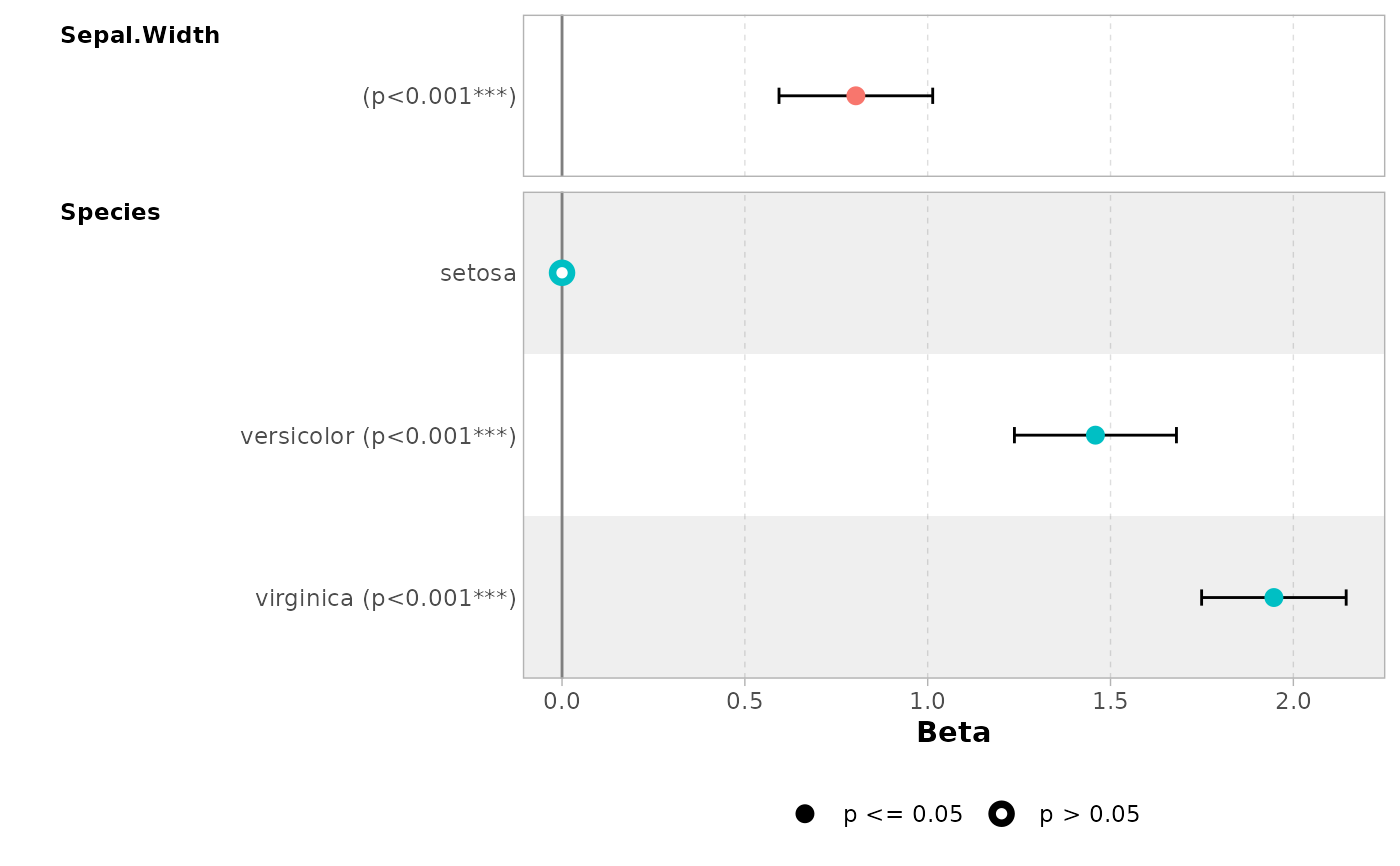ggcoef_table(mod)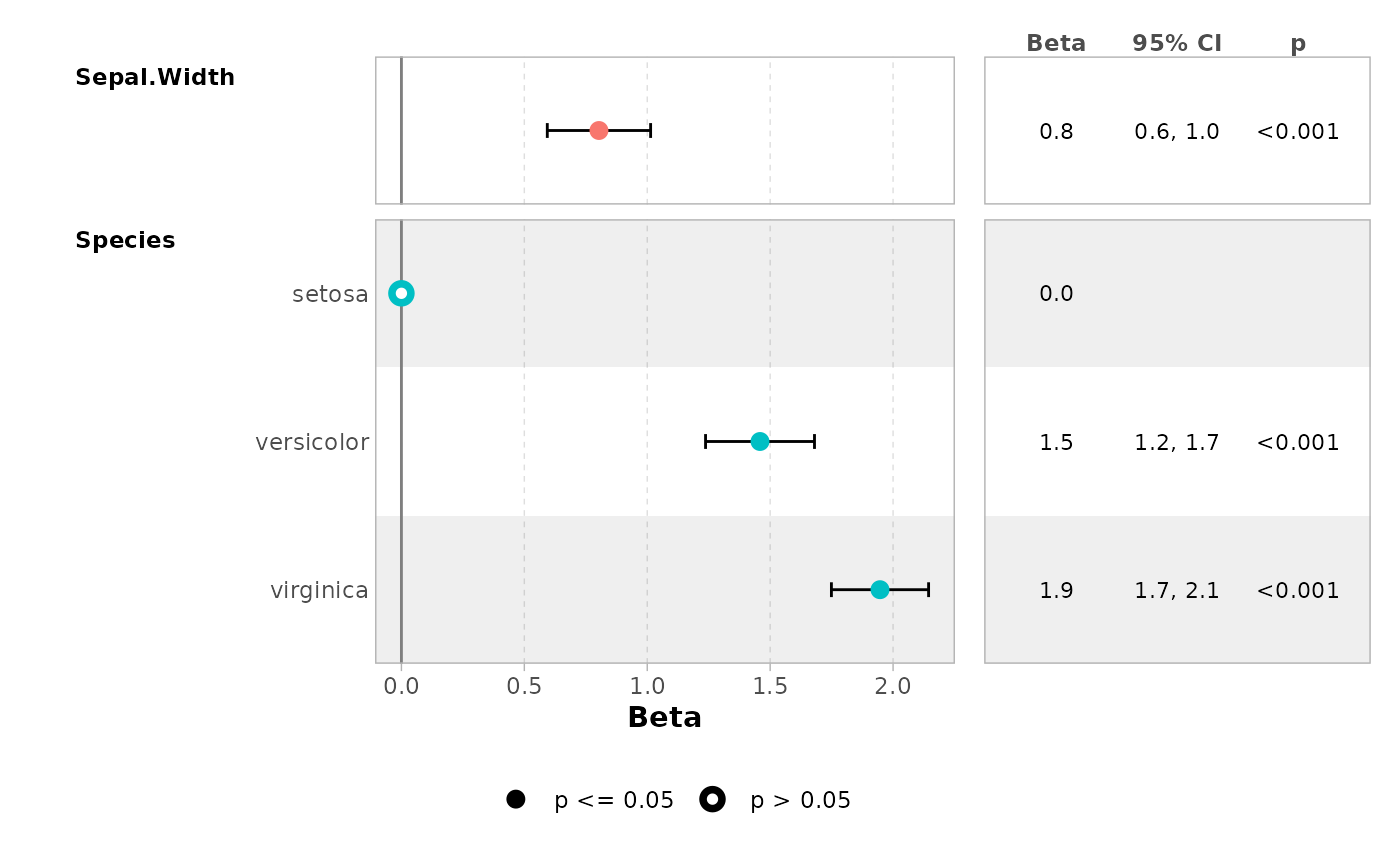# \donttest{
ggcoef_table(mod, table_stat = c("estimate", "ci"))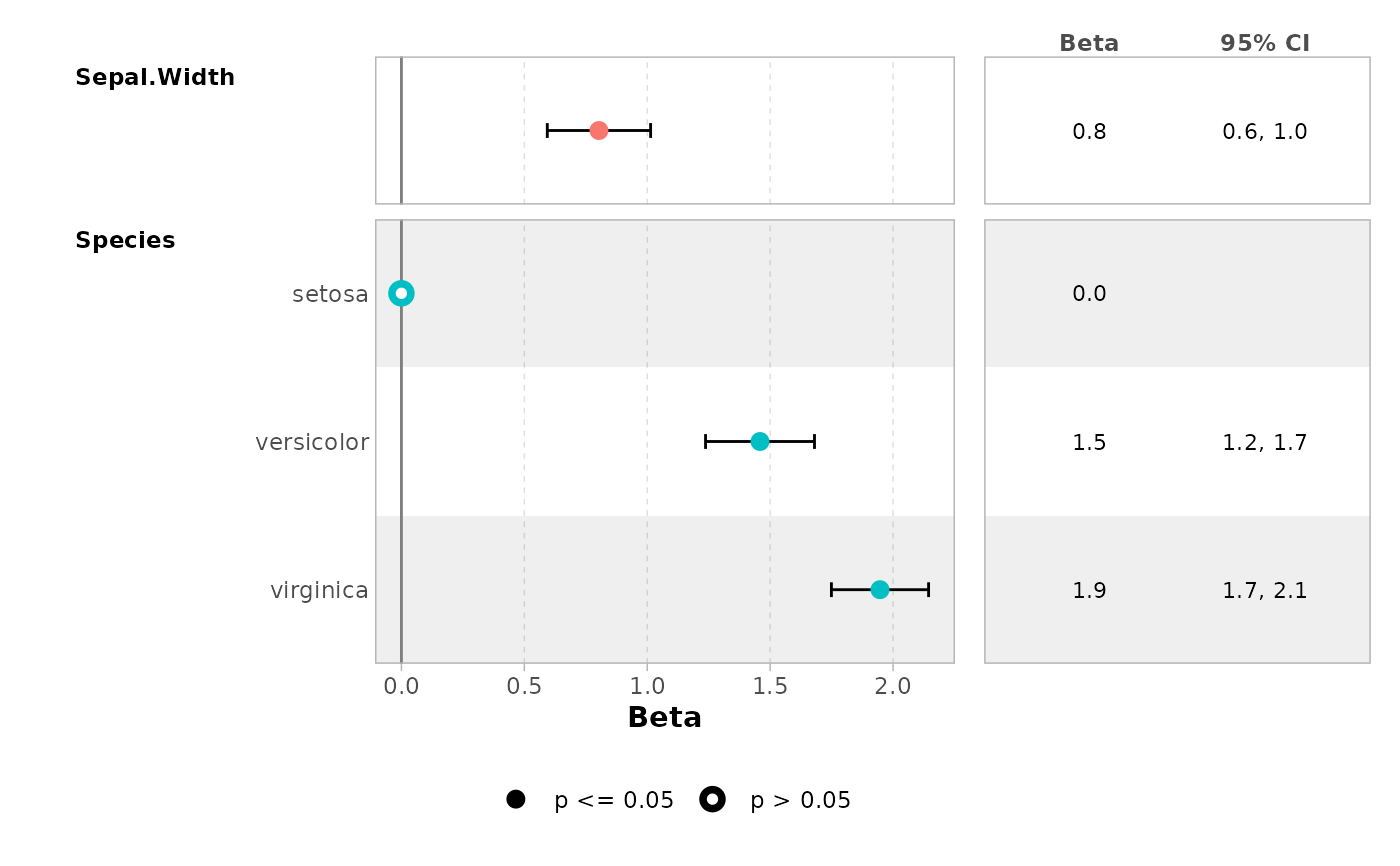ggcoef_table(
mod,
table_stat_label = list(
estimate = scales::label_number(.001)
)
)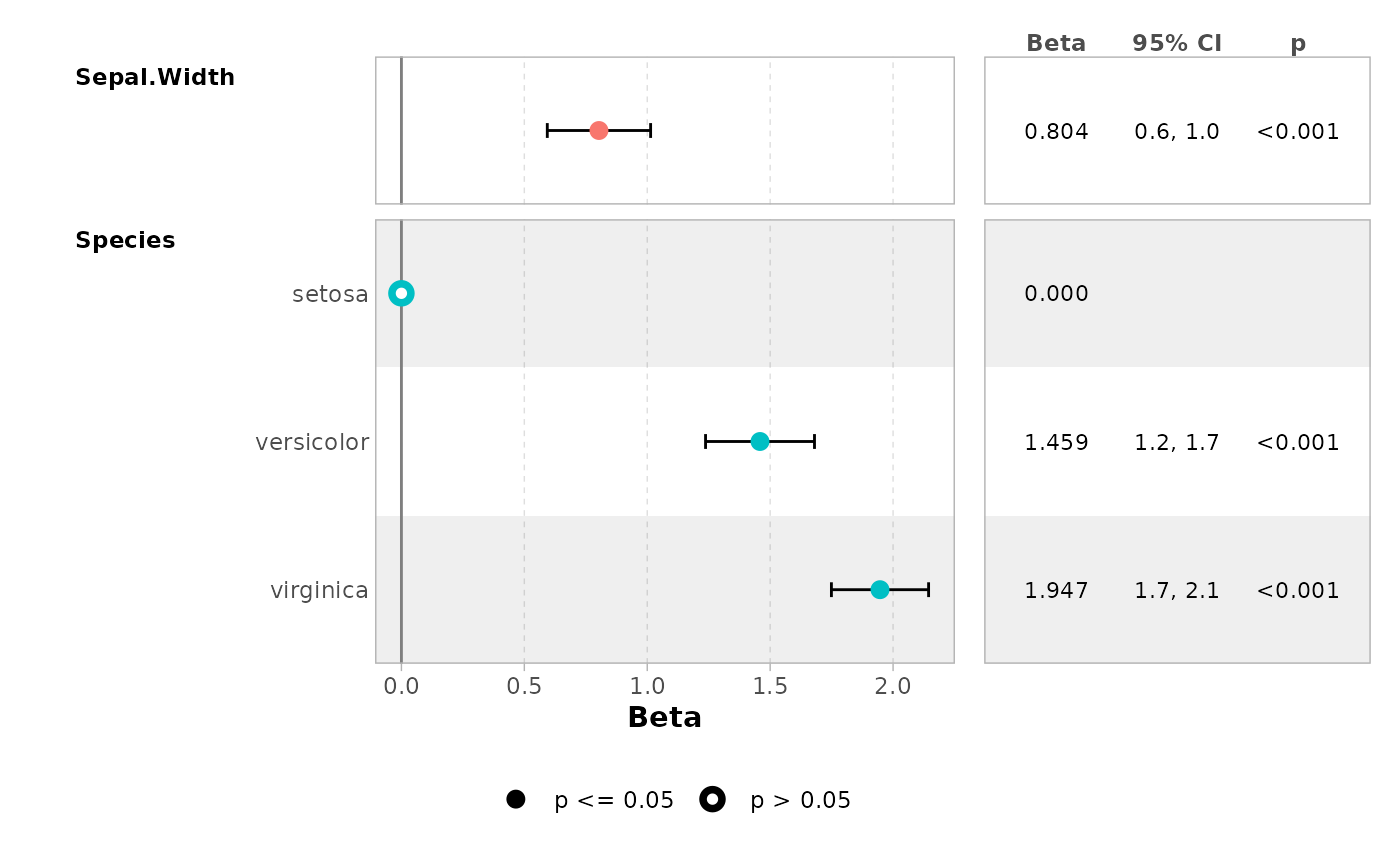ggcoef_table(mod, table_text_size = 5, table_witdhs = c(1, 1))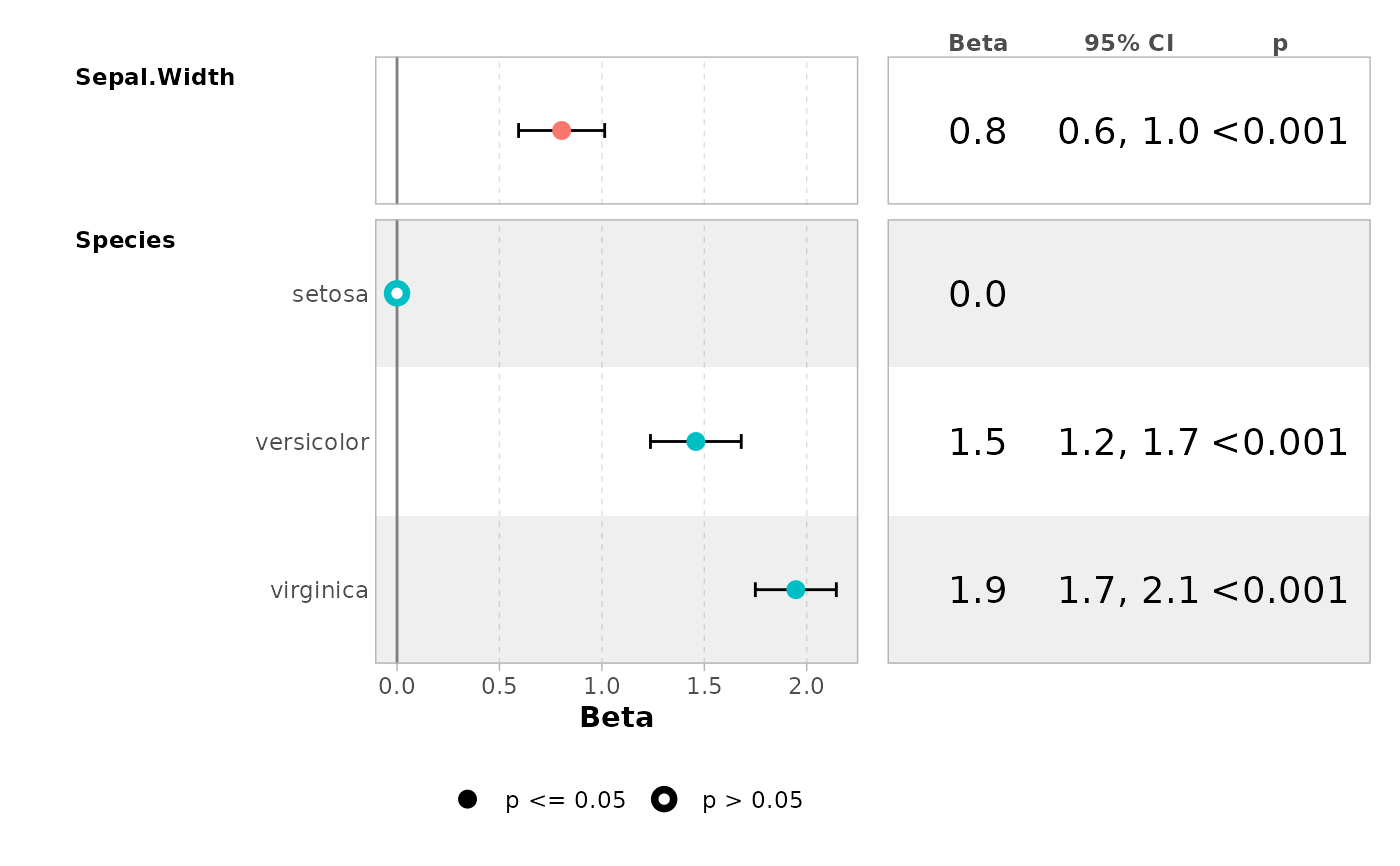# a logistic regression example
d_titanic <- as.data.frame(Titanic)
d_titanic$Survived <- factor(d_titanic$Survived, c("No", "Yes"))
mod_titanic <- glm(
Survived ~ Sex * Age + Class,
weights = Freq,
data = d_titanic,
family = binomial
)

# use 'exponentiate = TRUE' to get the Odds Ratio
ggcoef_model(mod_titanic, exponentiate = TRUE)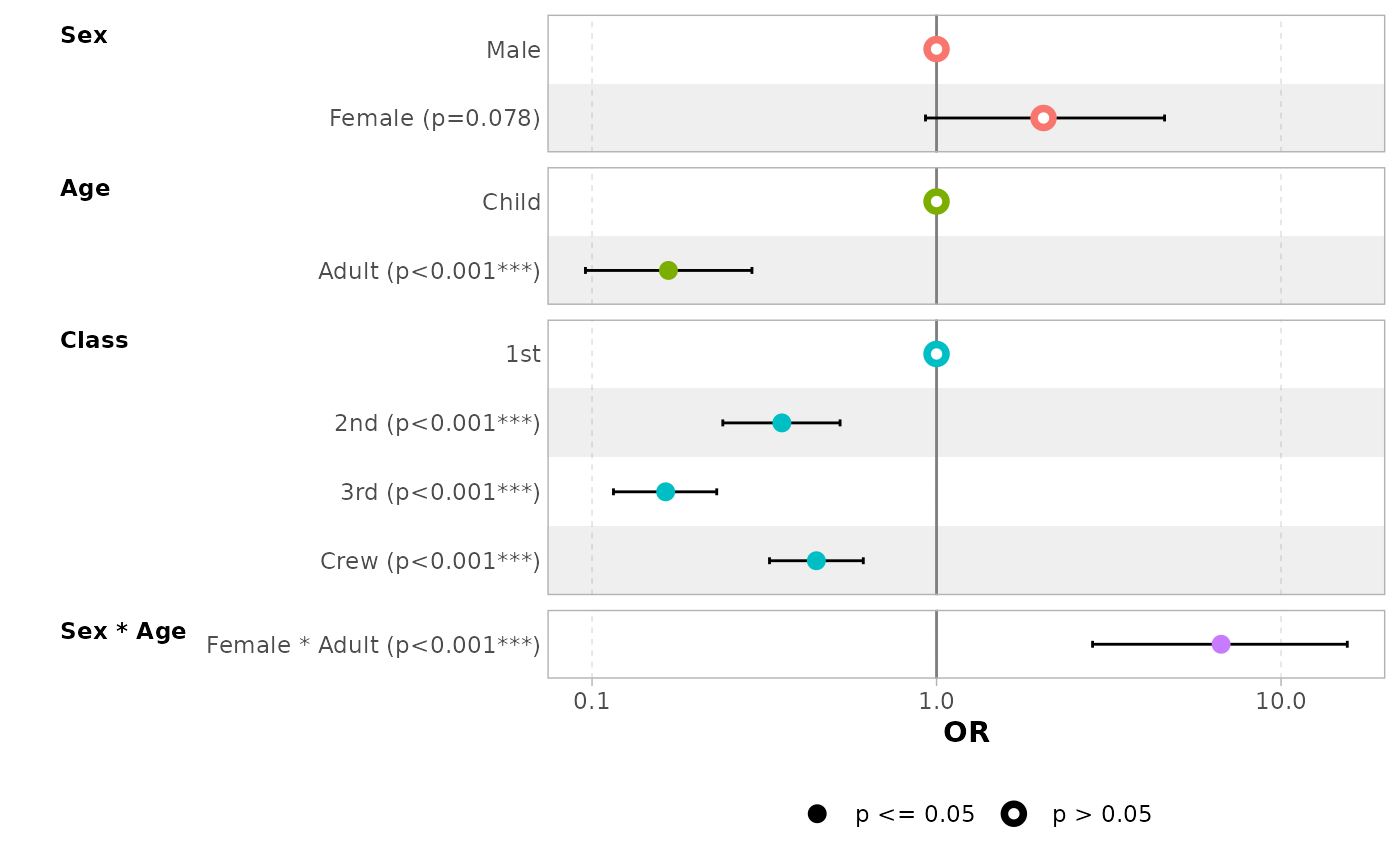ggcoef_table(mod_titanic, exponentiate = TRUE)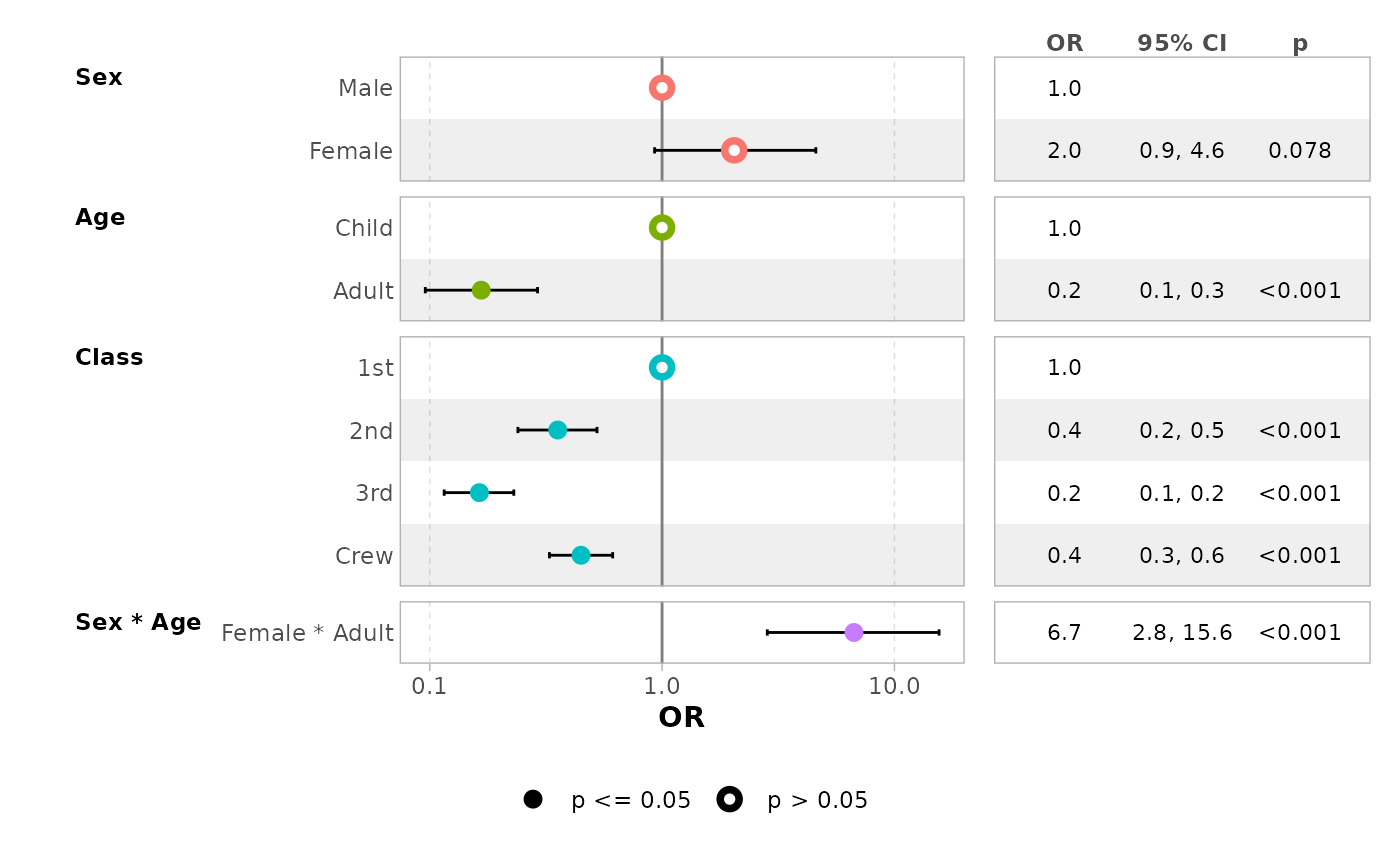# display intercepts
ggcoef_model(mod_titanic, exponentiate = TRUE, intercept = TRUE)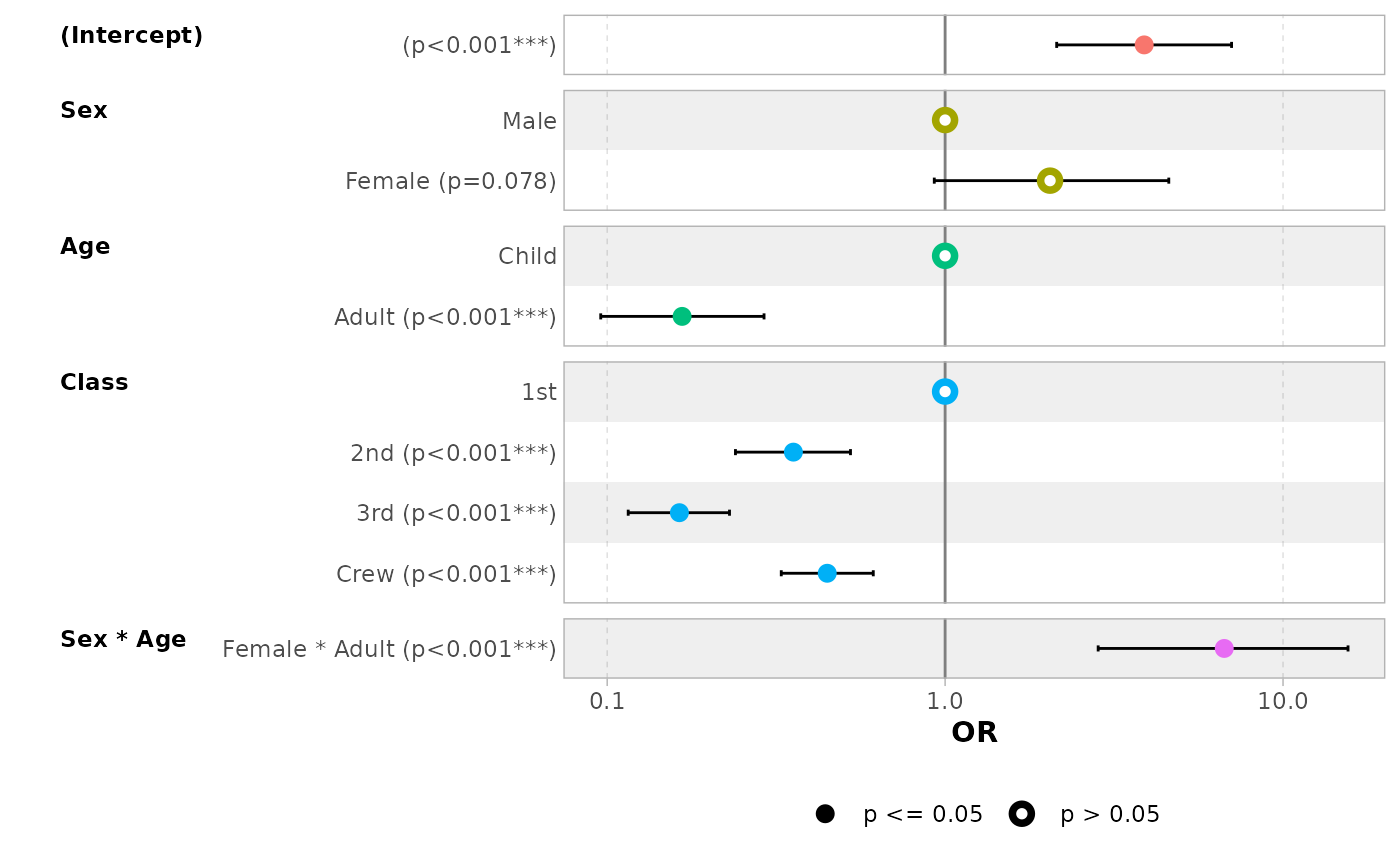# customize terms labels
ggcoef_model(
mod_titanic,
exponentiate = TRUE,
show_p_values = FALSE,
signif_stars = FALSE,
categorical_terms_pattern = "{level} (ref: {reference_level})",
interaction_sep = " x "
) +
ggplot2::scale_y_discrete(labels = scales::label_wrap(15))
#> Scale for y is already present.
#> Adding another scale for y, which will replace the existing scale.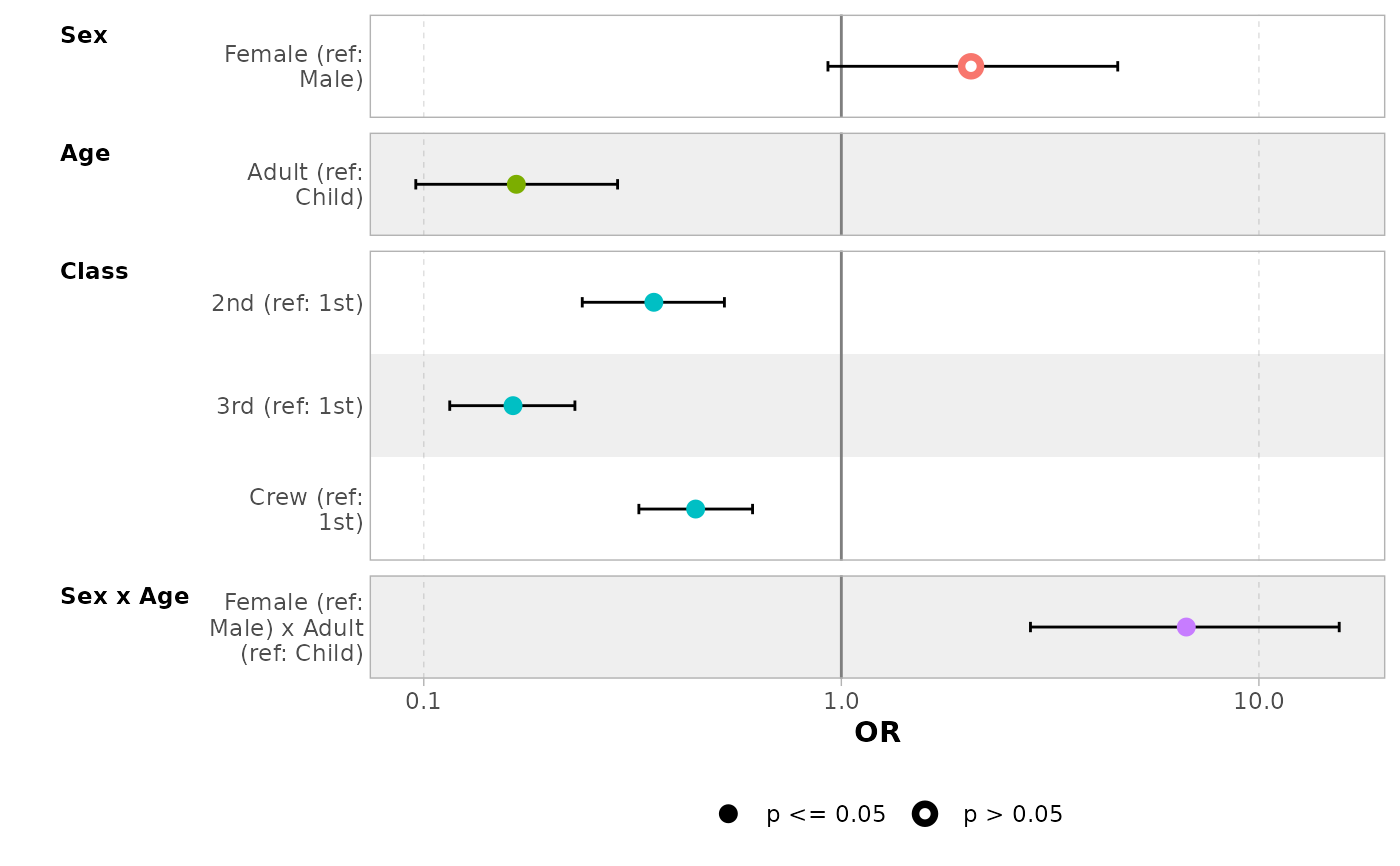# display only a subset of terms
ggcoef_model(mod_titanic, exponentiate = TRUE, include = c("Age", "Class"))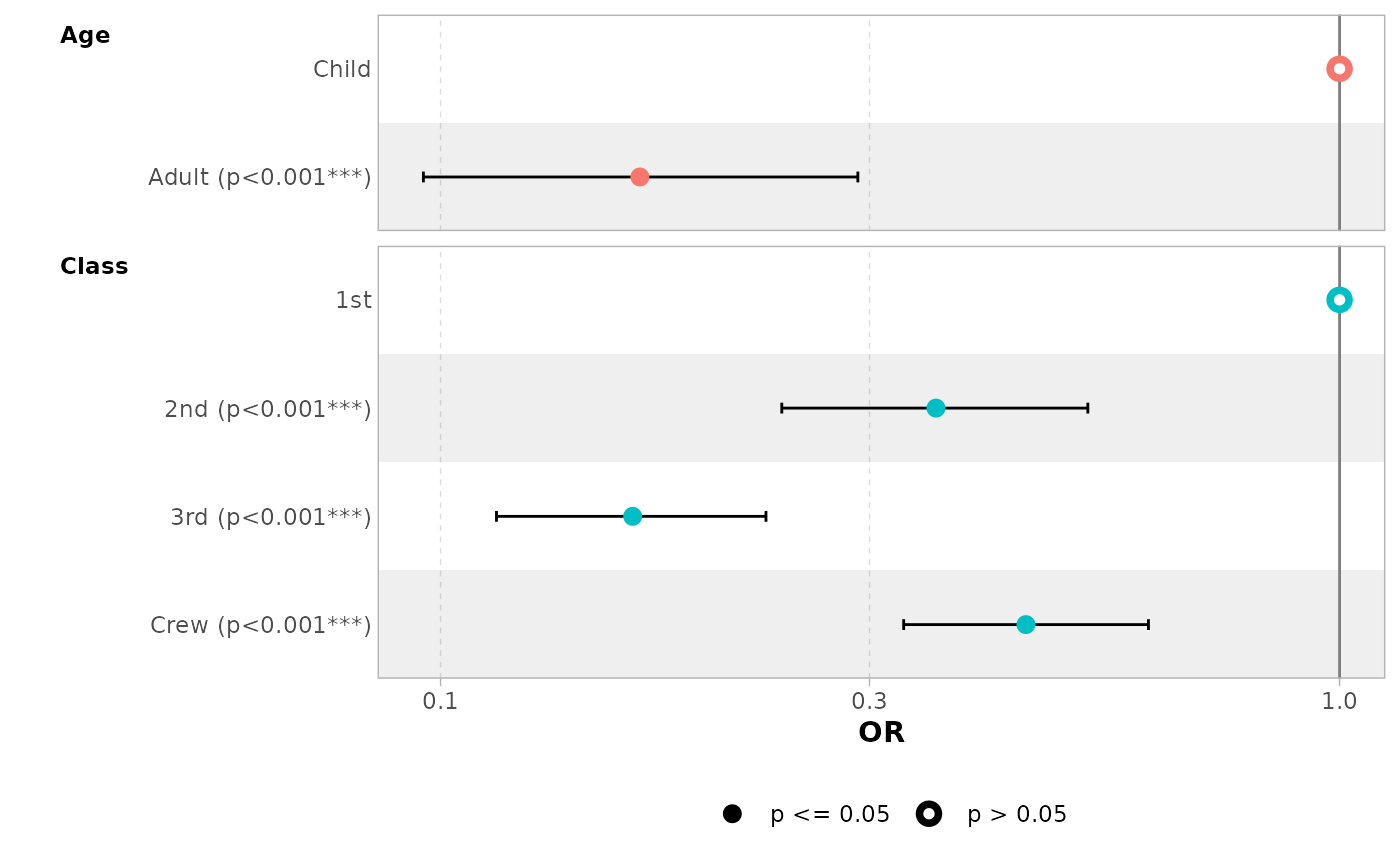# do not change points' shape based on significance
ggcoef_model(mod_titanic, exponentiate = TRUE, significance = NULL)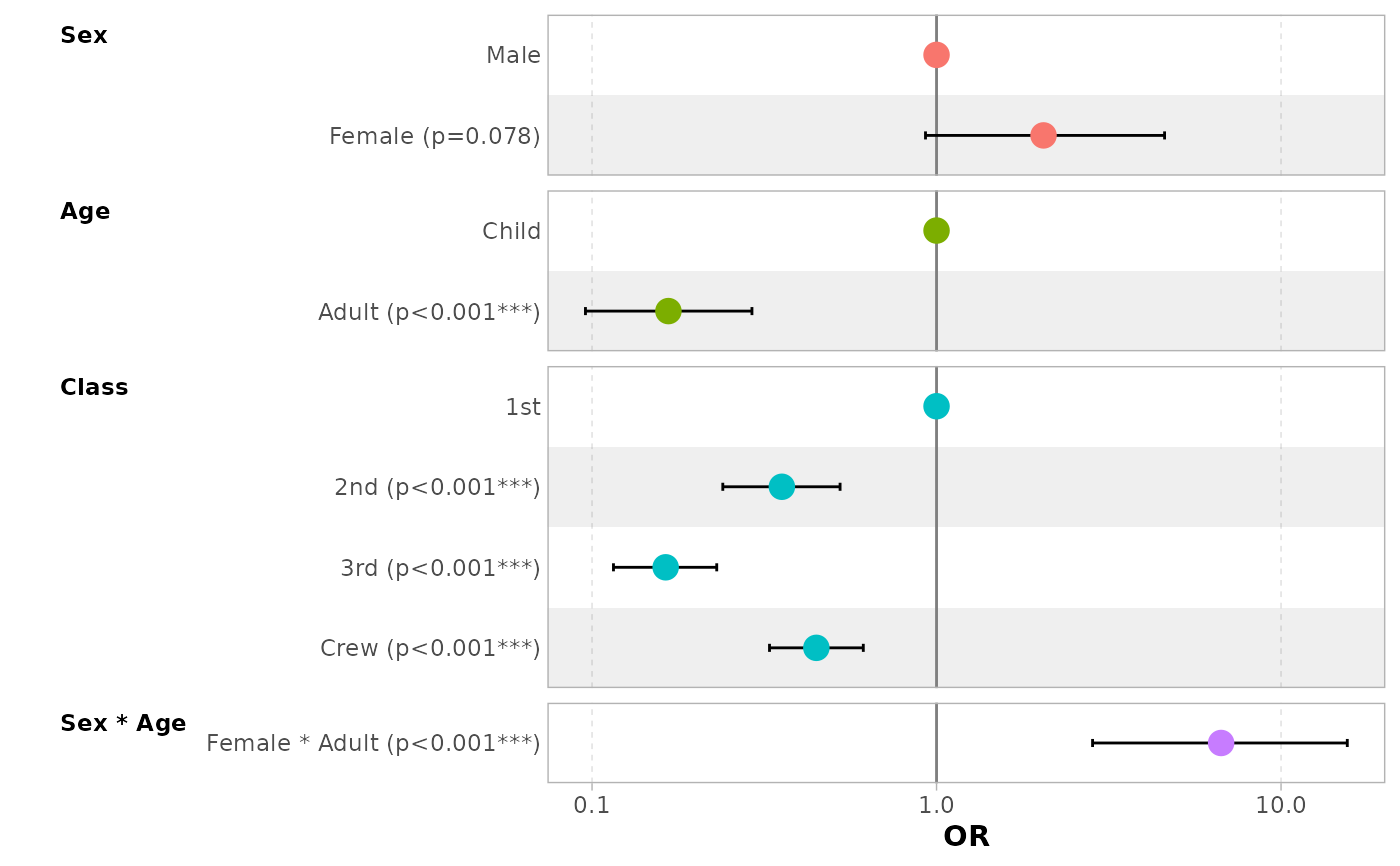# a black and white version
ggcoef_model(
mod_titanic,
exponentiate = TRUE,
colour = NULL, stripped_rows = FALSE
)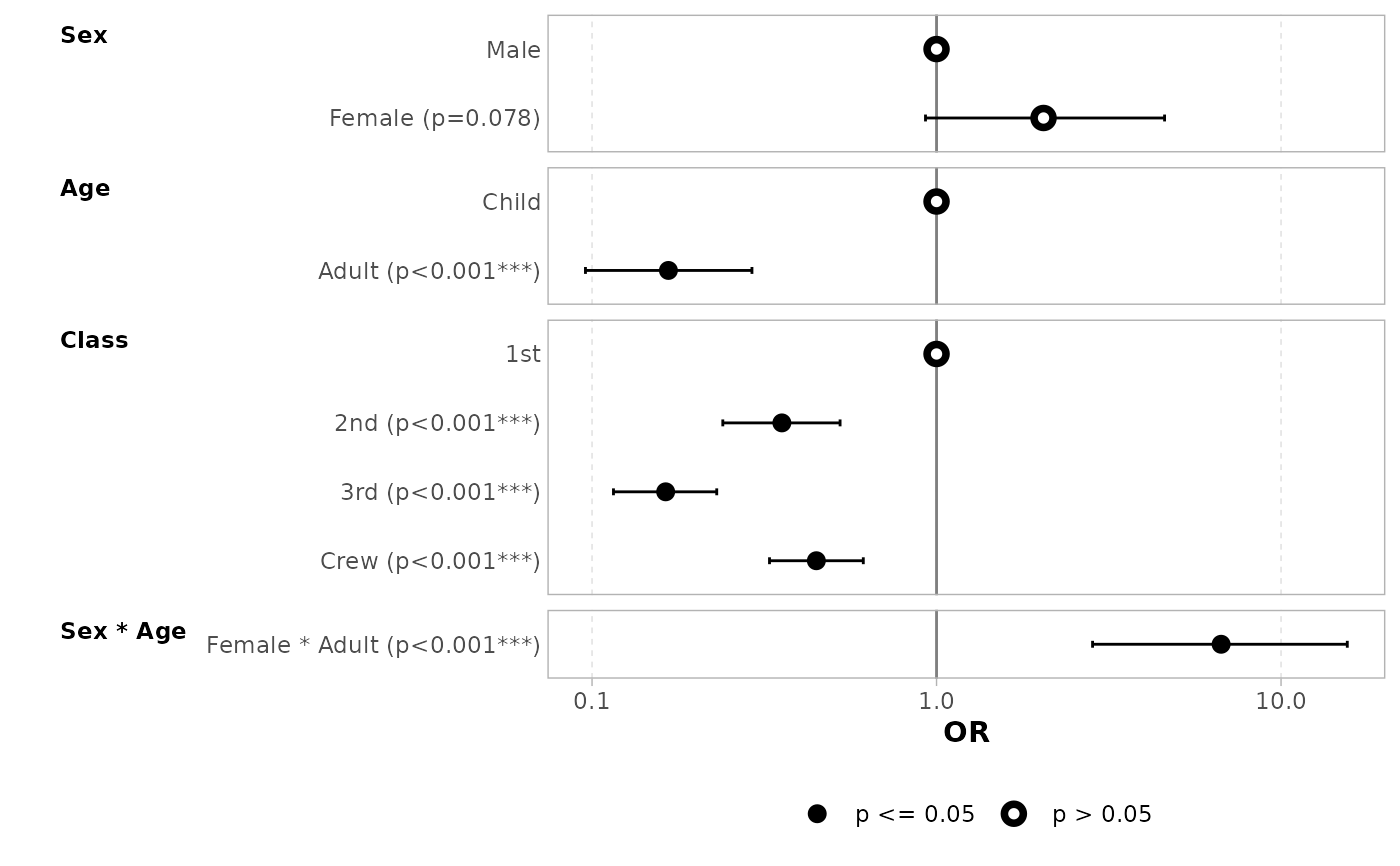# show dichotomous terms on one row
ggcoef_model(
mod_titanic,
exponentiate = TRUE,
no_reference_row = broom.helpers::all_dichotomous(),
categorical_terms_pattern =
"{ifelse(dichotomous, paste0(level, ' / ', reference_level), level)}",
show_p_values = FALSE
)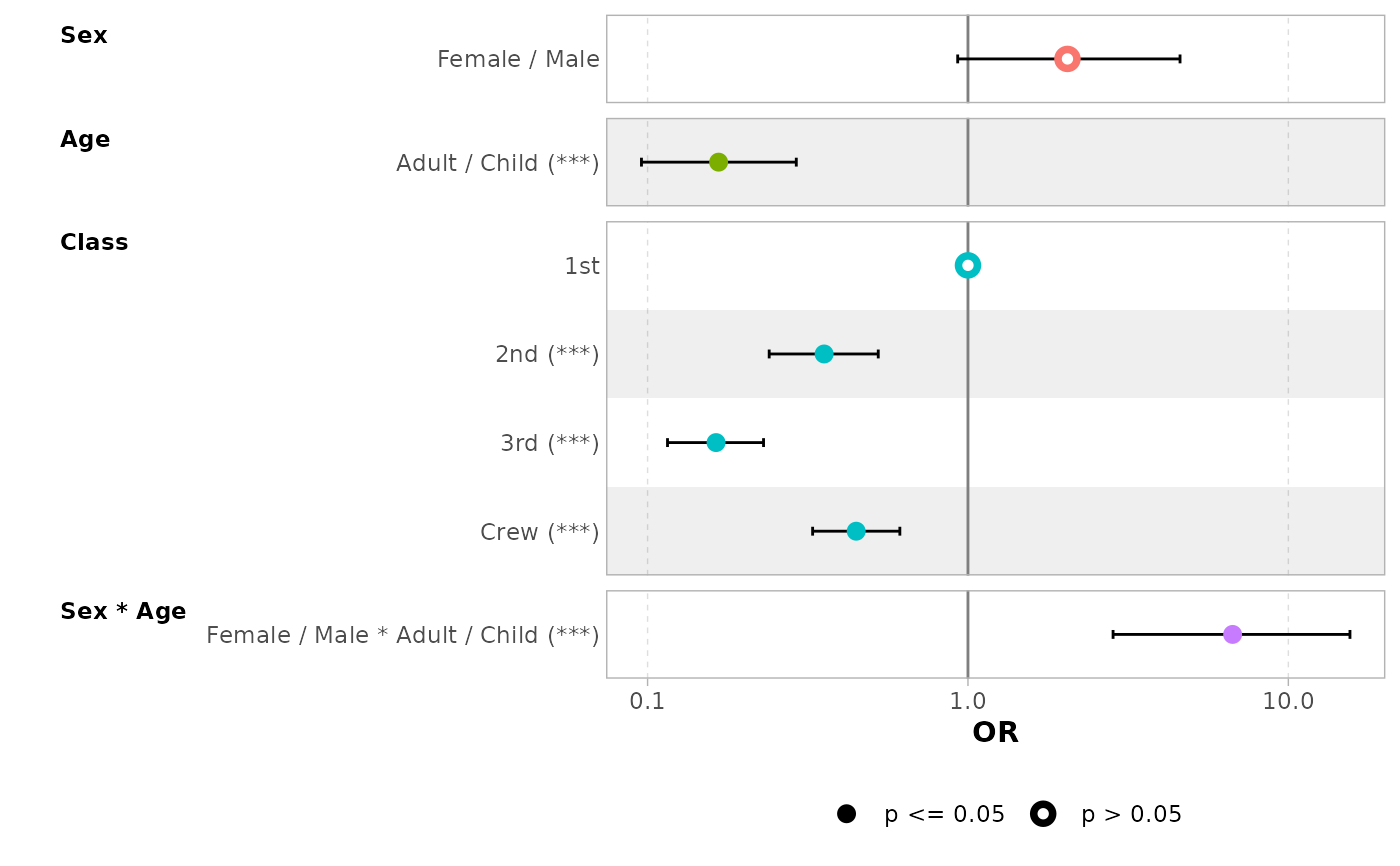# }

# \donttest{
data(tips, package = "reshape")
mod_simple <- lm(tip ~ day + time + total_bill, data = tips)
ggcoef_model(mod_simple)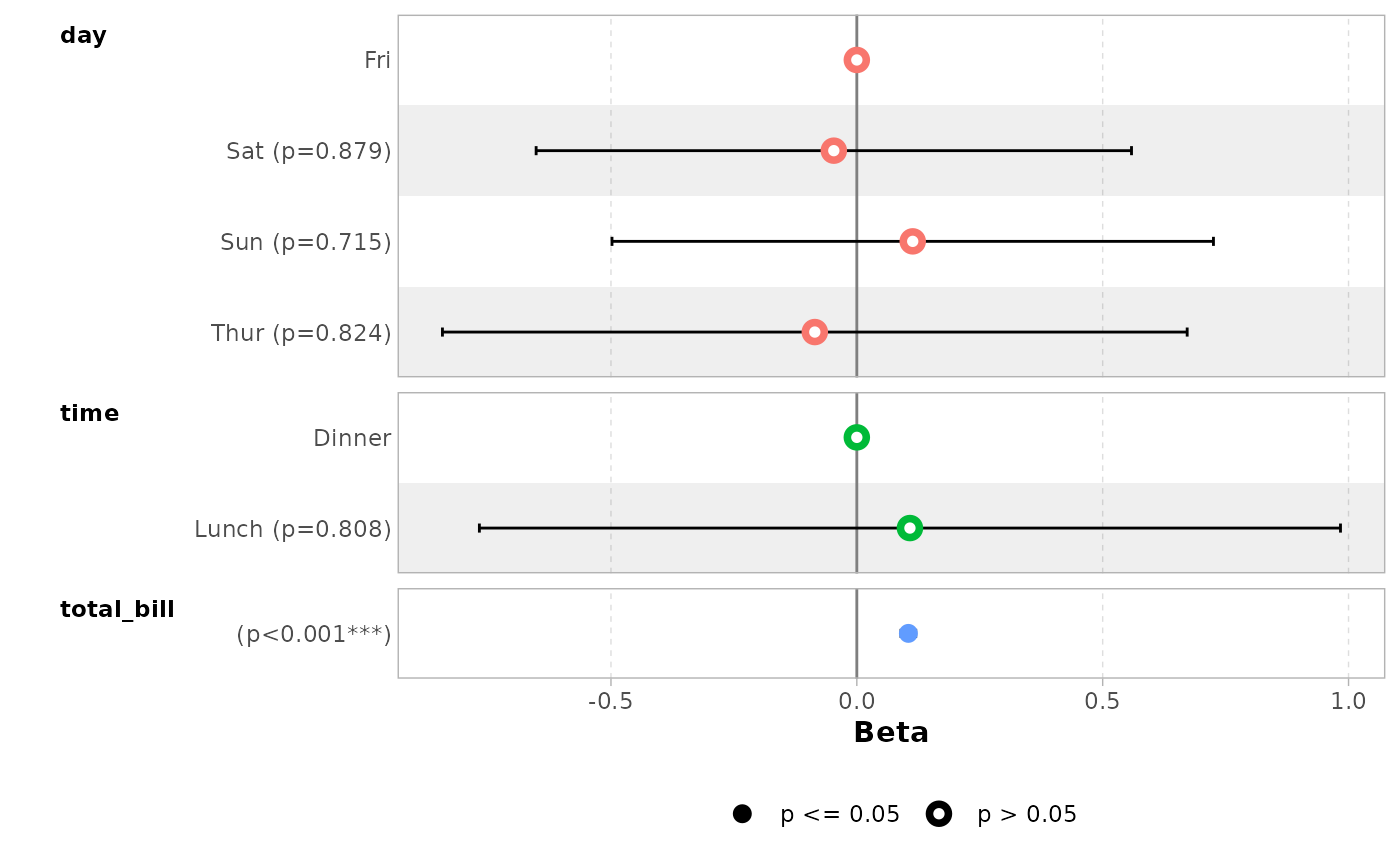# custom variable labels
# you can use the labelled package to define variable labels
# before computing model
if (requireNamespace("labelled")) {
tips_labelled <- tips %>%
labelled::set_variable_labels(
day = "Day of the week",
time = "Lunch or Dinner",
total_bill = "Bill's total"
)
mod_labelled <- lm(tip ~ day + time + total_bill, data = tips_labelled)
ggcoef_model(mod_labelled)
}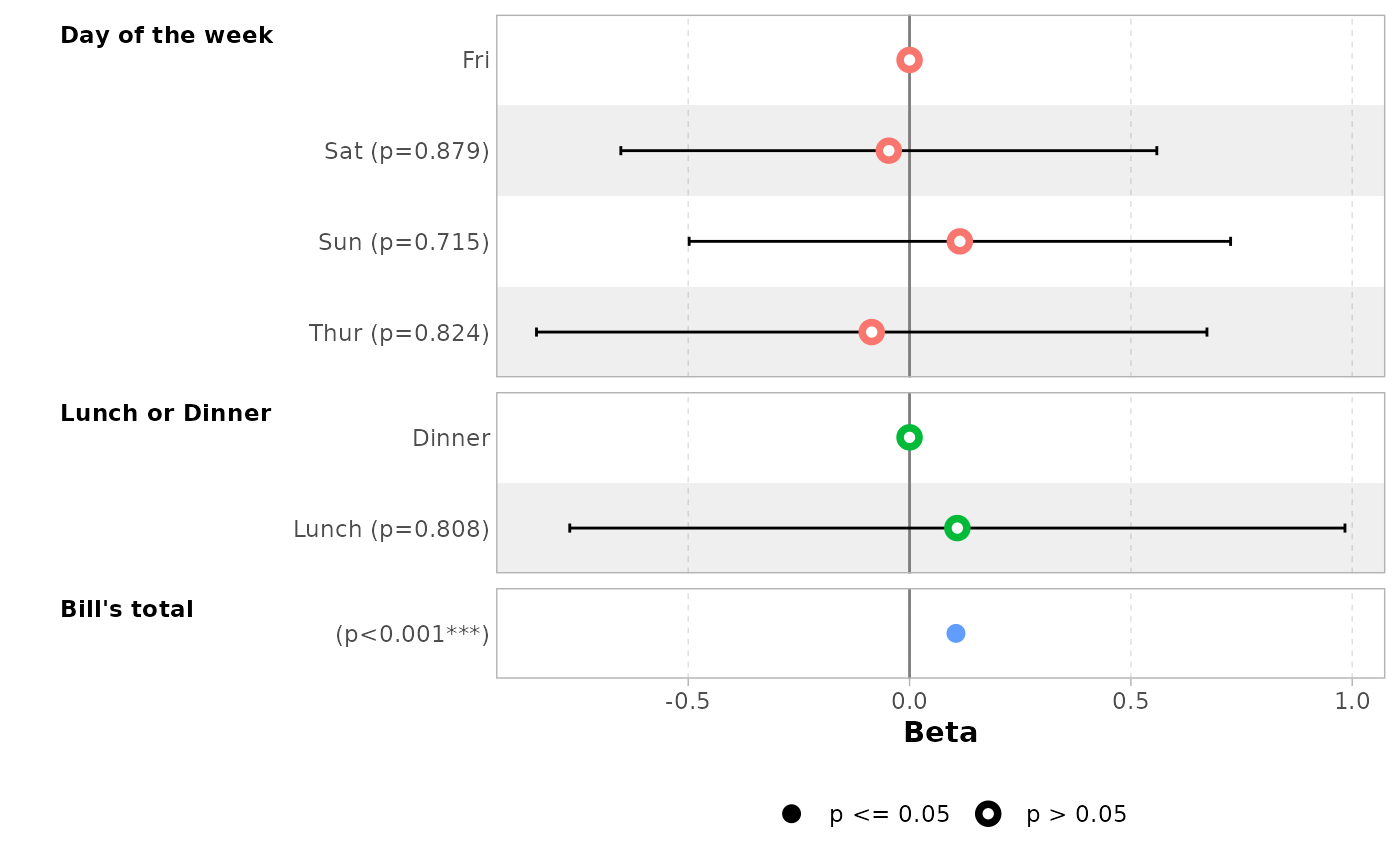# you can provide custom variable labels with 'variable_labels'
ggcoef_model(
mod_simple,
variable_labels = c(
day = "Week day",
time = "Time (lunch or dinner ?)",
total_bill = "Total of the bill"
)
)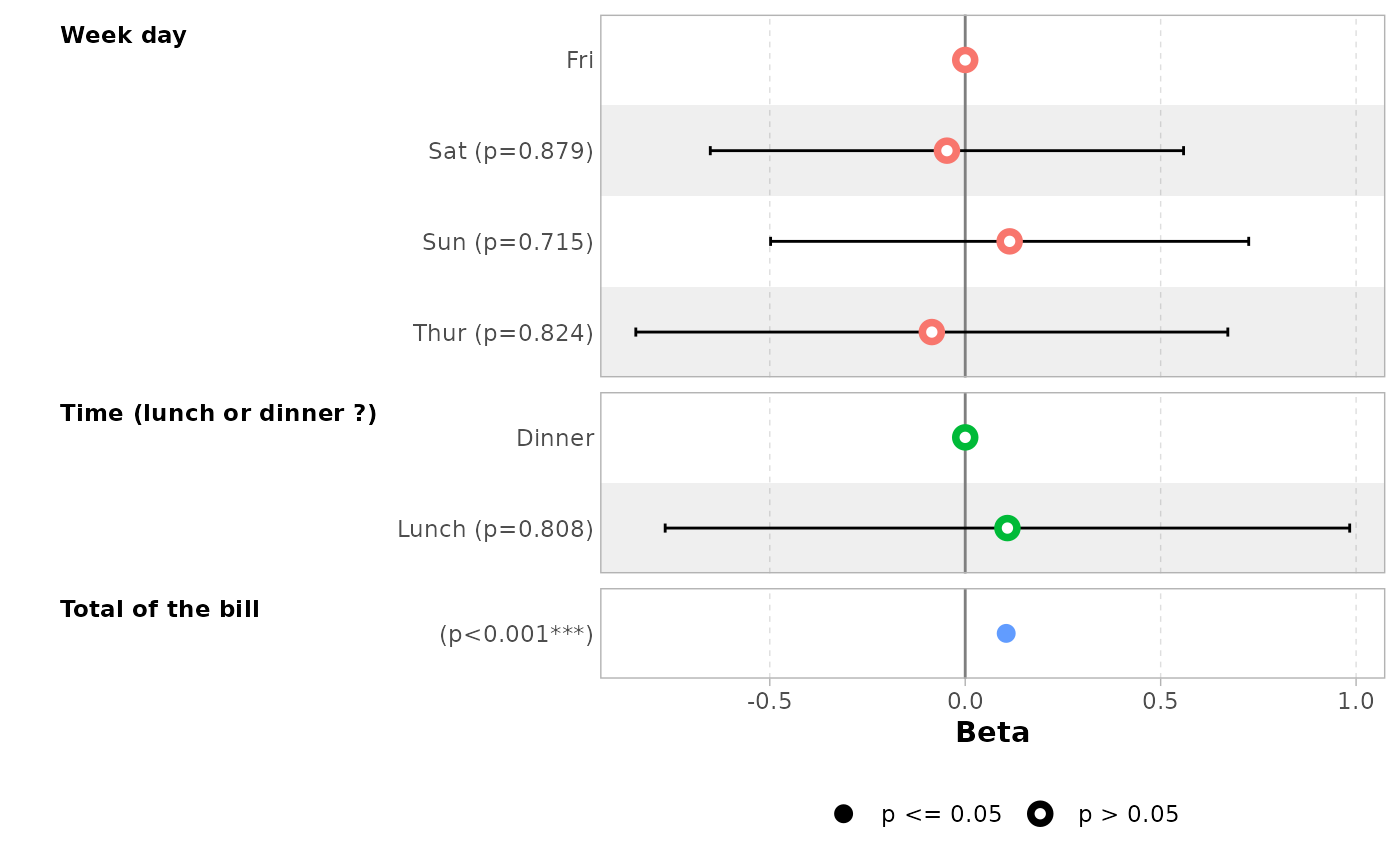# if labels are too long, you can use 'facet_labeller' to wrap them
ggcoef_model(
mod_simple,
variable_labels = c(
day = "Week day",
time = "Time (lunch or dinner ?)",
total_bill = "Total of the bill"
),
facet_labeller = ggplot2::label_wrap_gen(10)
)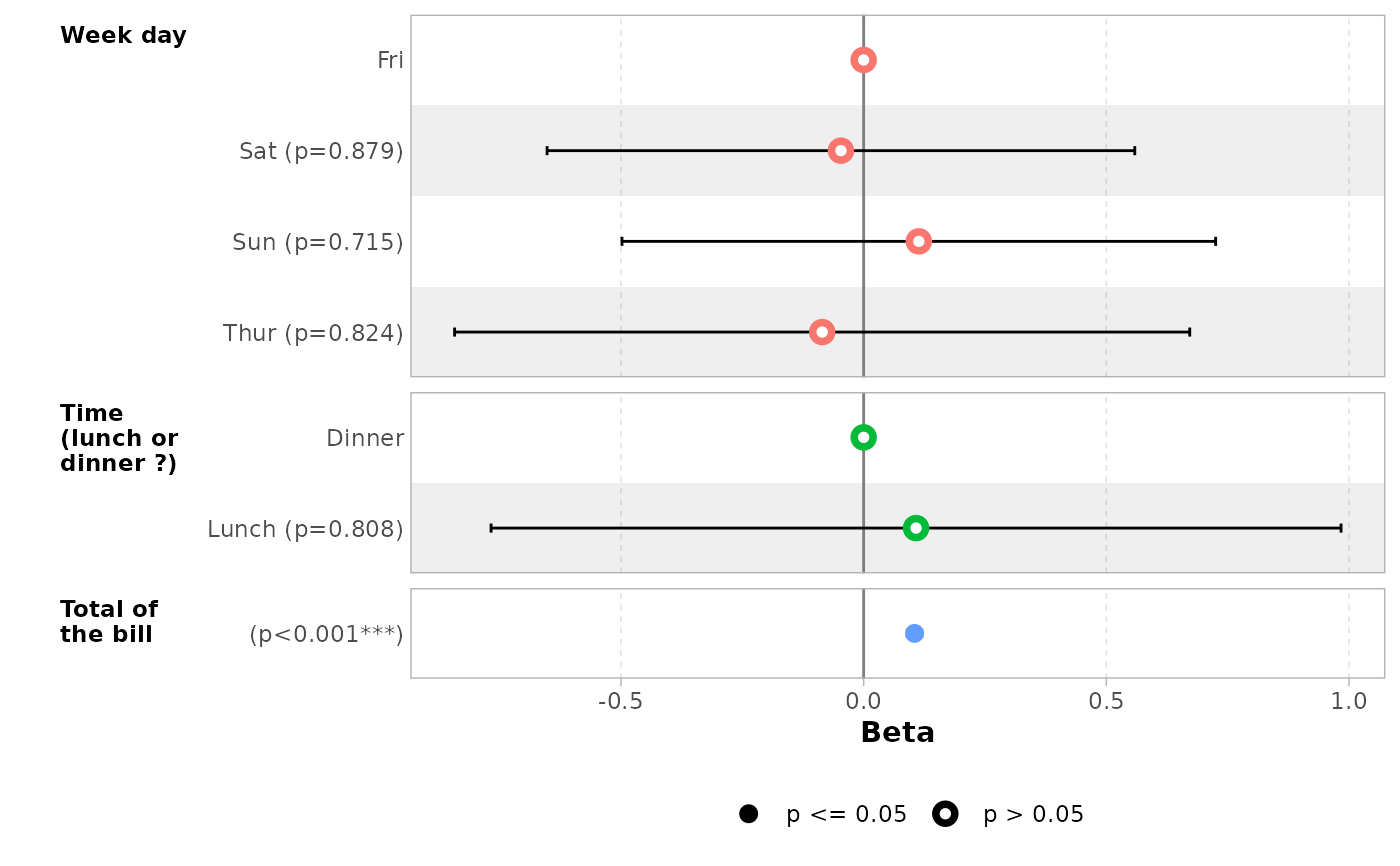# do not display variable facets but add colour guide
ggcoef_model(mod_simple, facet_row = NULL, colour_guide = TRUE)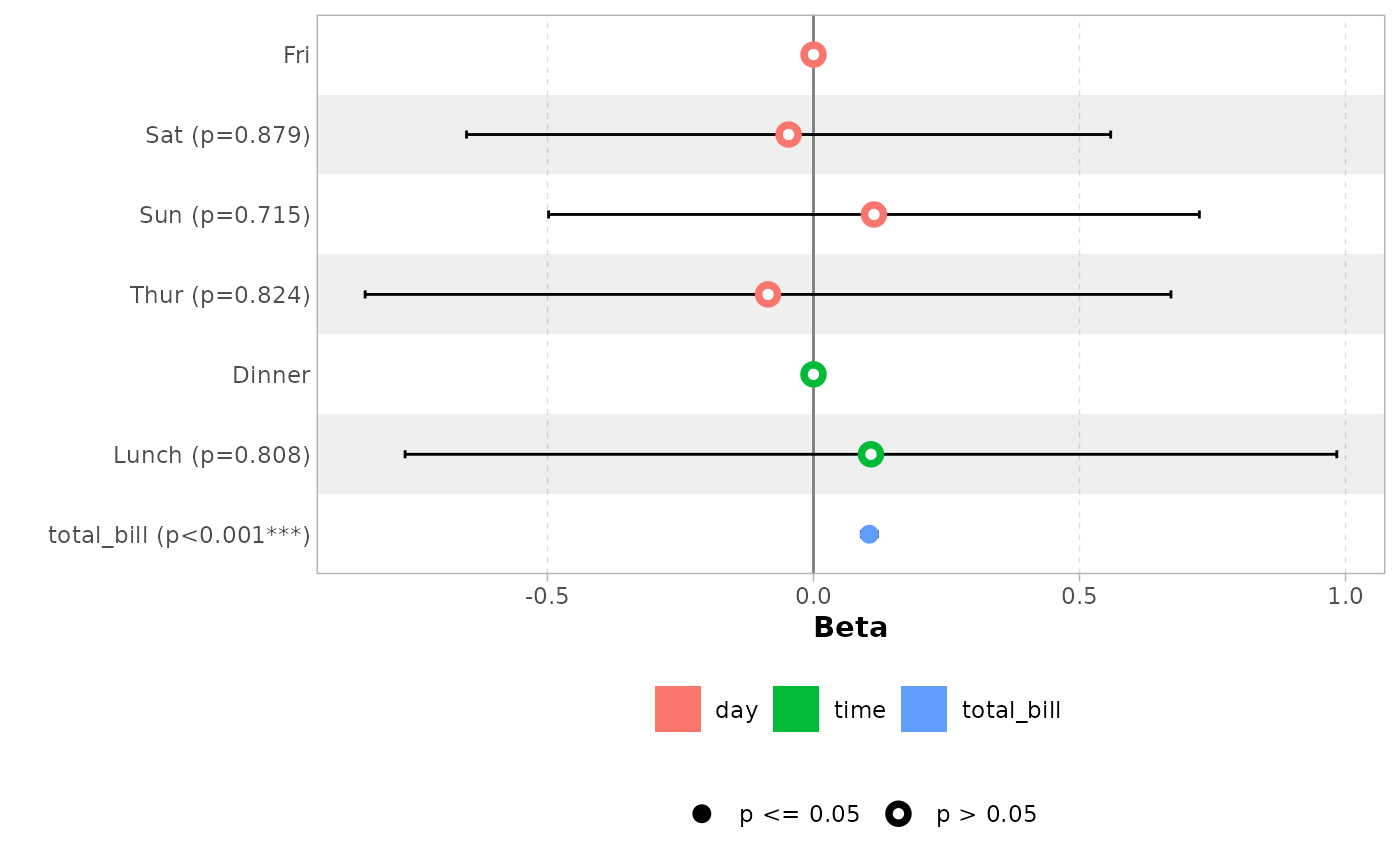# works also with with polynomial terms
mod_poly <- lm(
tip ~ poly(total_bill, 3) + day,
data = tips,
)
ggcoef_model(mod_poly)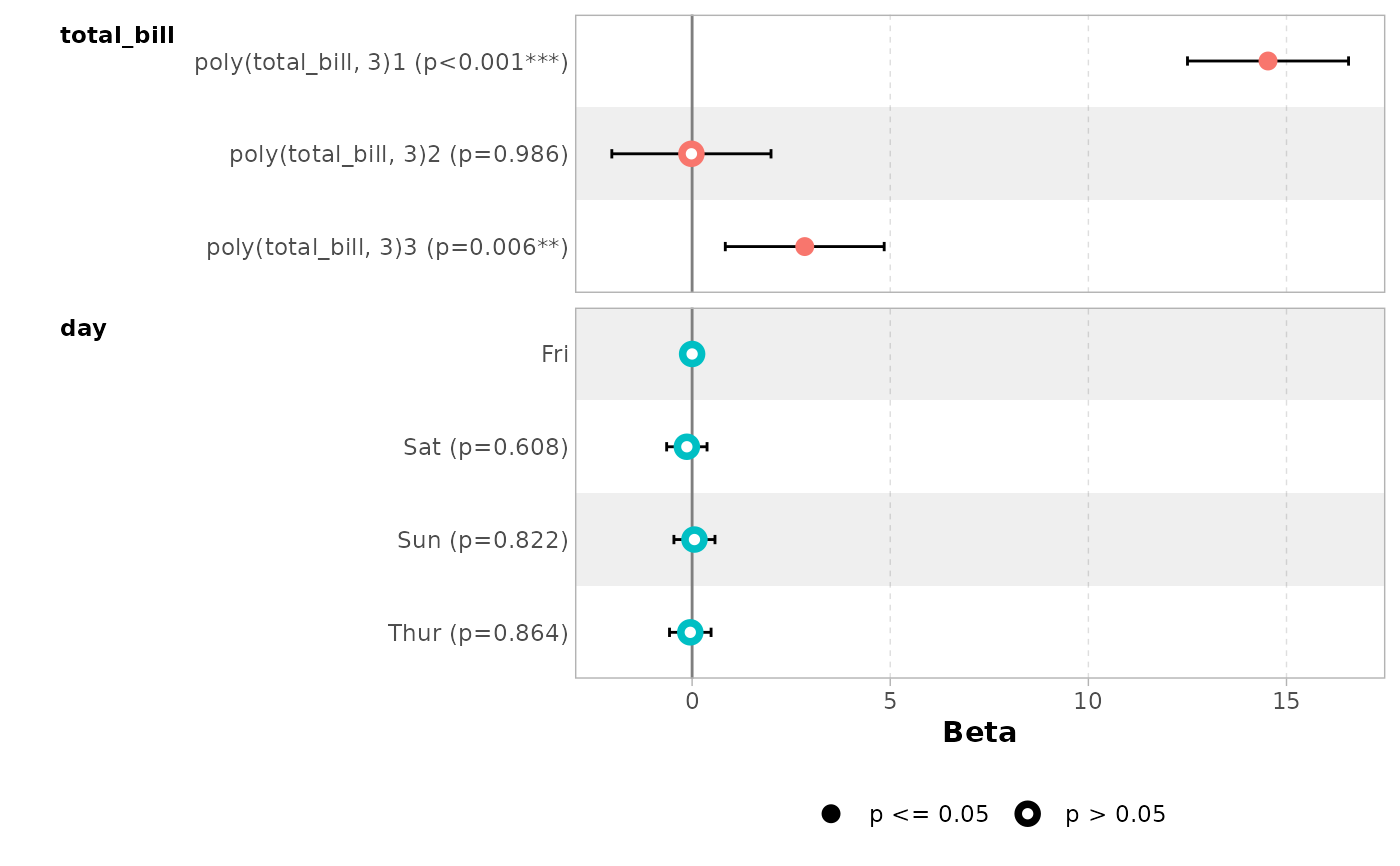# or with different type of contrasts
# for sum contrasts, the value of the reference term is computed
if (requireNamespace("emmeans")) {
mod2 <- lm(
tip ~ day + time + sex,
data = tips,
contrasts = list(time = contr.sum, day = contr.treatment(4, base = 3))
)
ggcoef_model(mod2)
}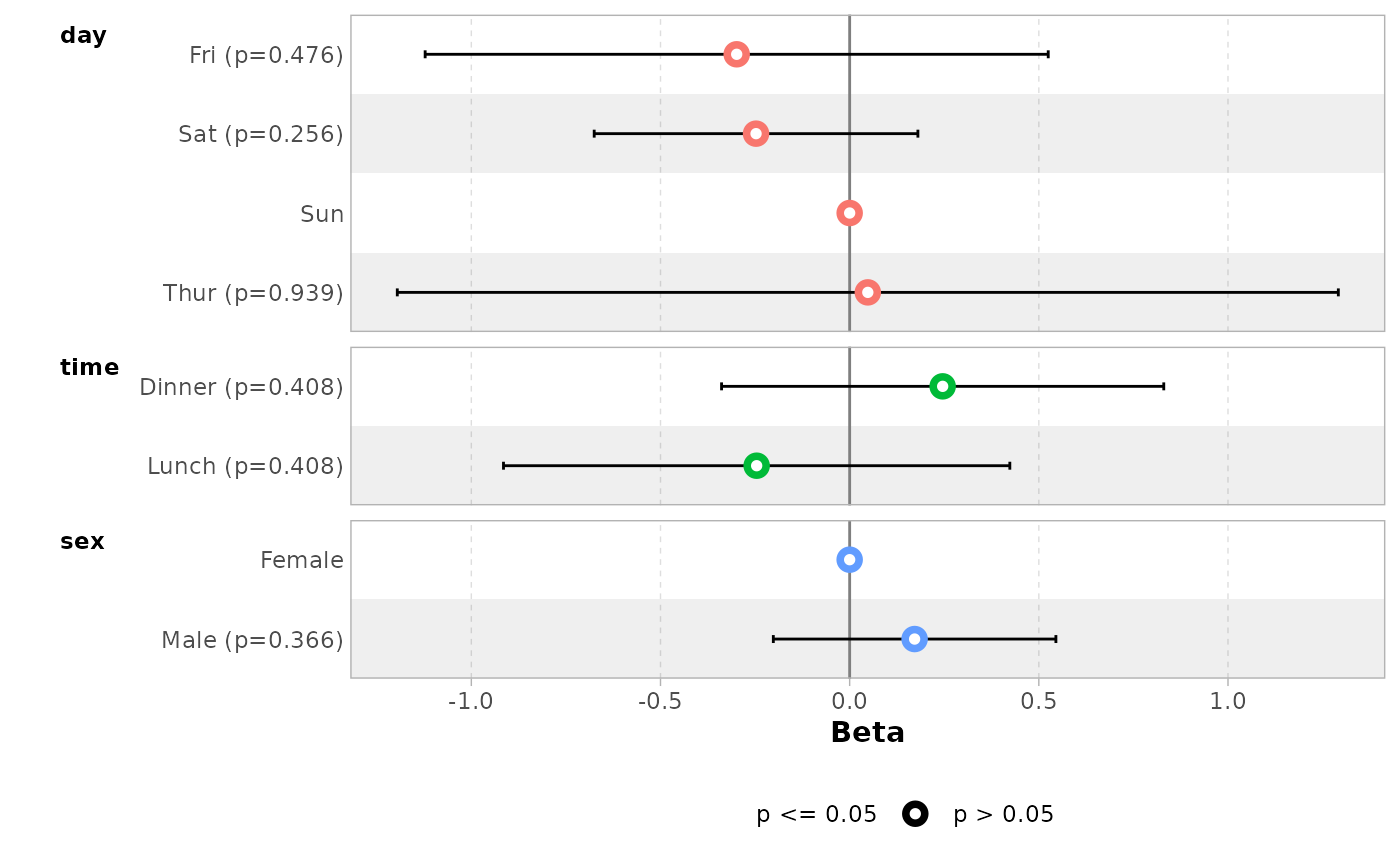# }
# \donttest{
# Use ggcoef_compare() for comparing several models on the same plot
mod1 <- lm(Fertility ~ ., data = swiss)
mod2 <- step(mod1, trace = 0)
mod3 <- lm(Fertility ~ Agriculture + Education * Catholic, data = swiss)
models <- list(
"Full model" = mod1,
"Simplified model" = mod2,
"With interaction" = mod3
)

ggcoef_compare(models)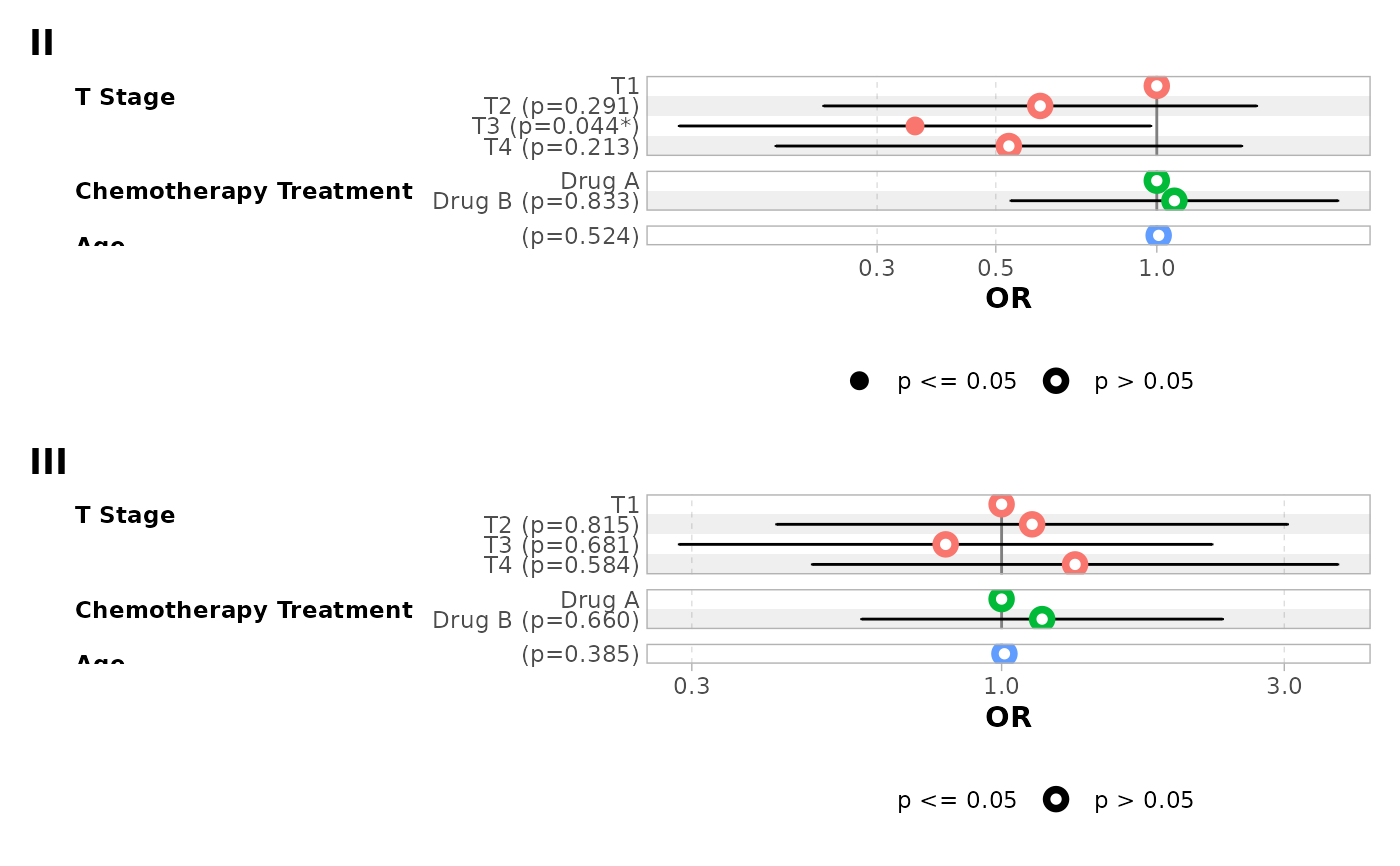ggcoef_compare(models, type = "faceted")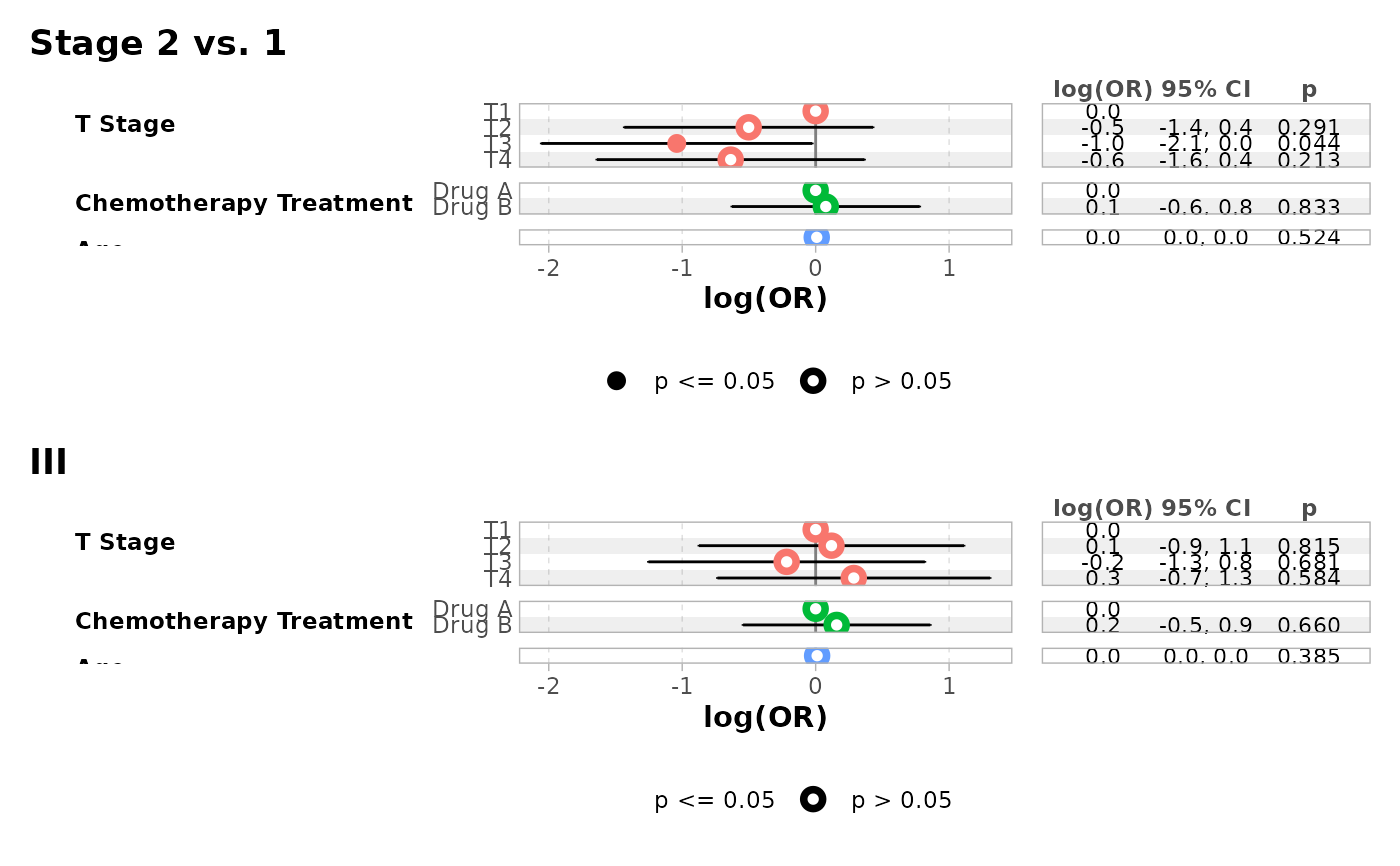# you can reverse the vertical position of the point by using a negative
# value for dodged_width (but it will produce some warnings)
ggcoef_compare(models, dodged_width = -.9)
#> Warning: position_dodge() requires non-overlapping x intervals
#> Warning: position_dodge() requires non-overlapping x intervals
#> Warning: position_dodge() requires non-overlapping x intervals
#> Warning: position_dodge() requires non-overlapping x intervals
#> Warning: position_dodge() requires non-overlapping x intervals
#> Warning: position_dodge() requires non-overlapping x intervals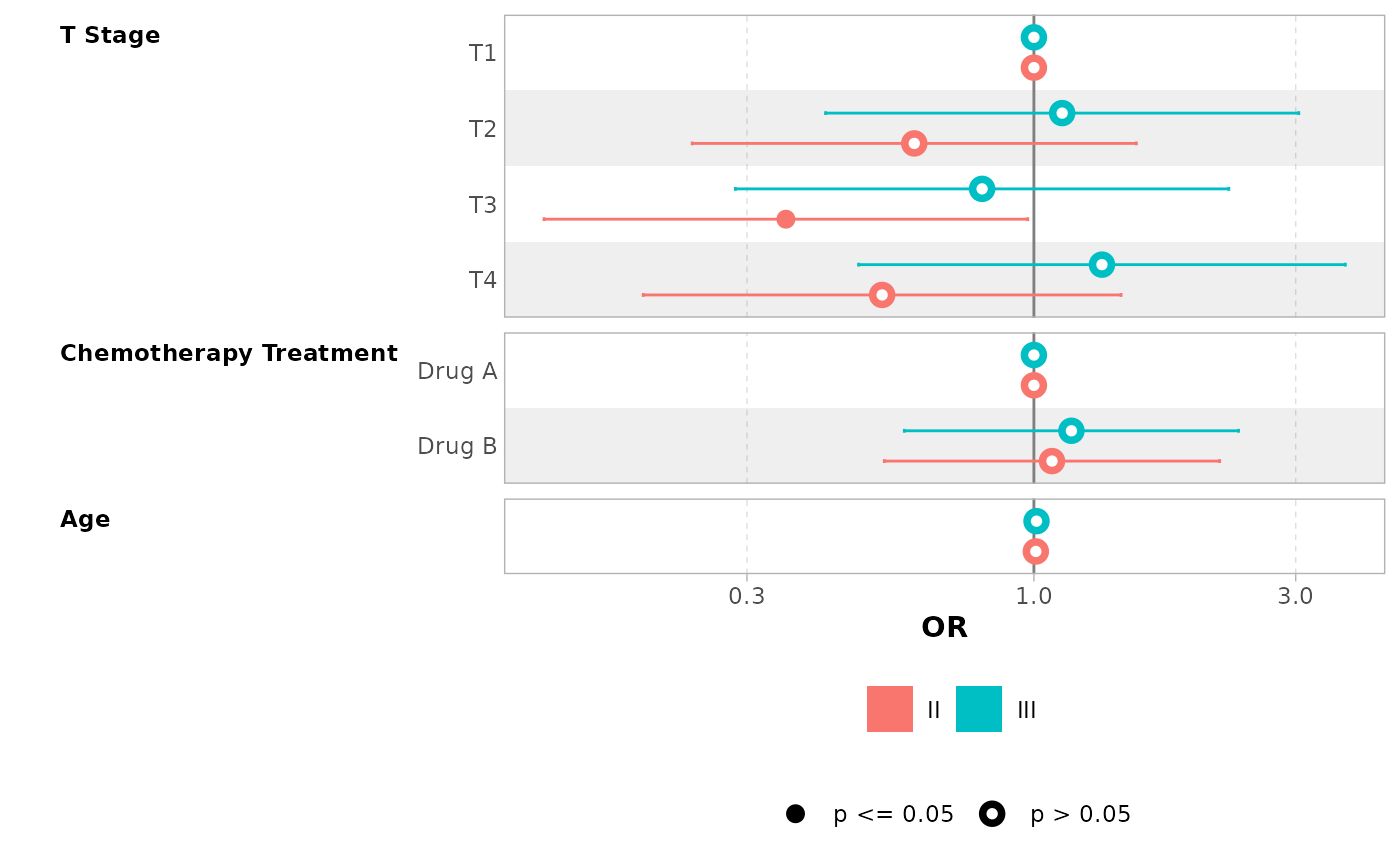# }

# \donttest{
# specific function for nnet::multinom models
mod <- nnet::multinom(Species ~ ., data = iris)
#> # weights:  18 (10 variable)
#> initial  value 164.791843
#> iter  10 value 16.177348
#> iter  20 value 7.111438
#> iter  30 value 6.182999
#> iter  40 value 5.984028
#> iter  50 value 5.961278
#> iter  60 value 5.954900
#> iter  70 value 5.951851
#> iter  80 value 5.950343
#> iter  90 value 5.949904
#> iter 100 value 5.949867
#> final  value 5.949867
#> stopped after 100 iterations
ggcoef_multinom(mod, exponentiate = TRUE)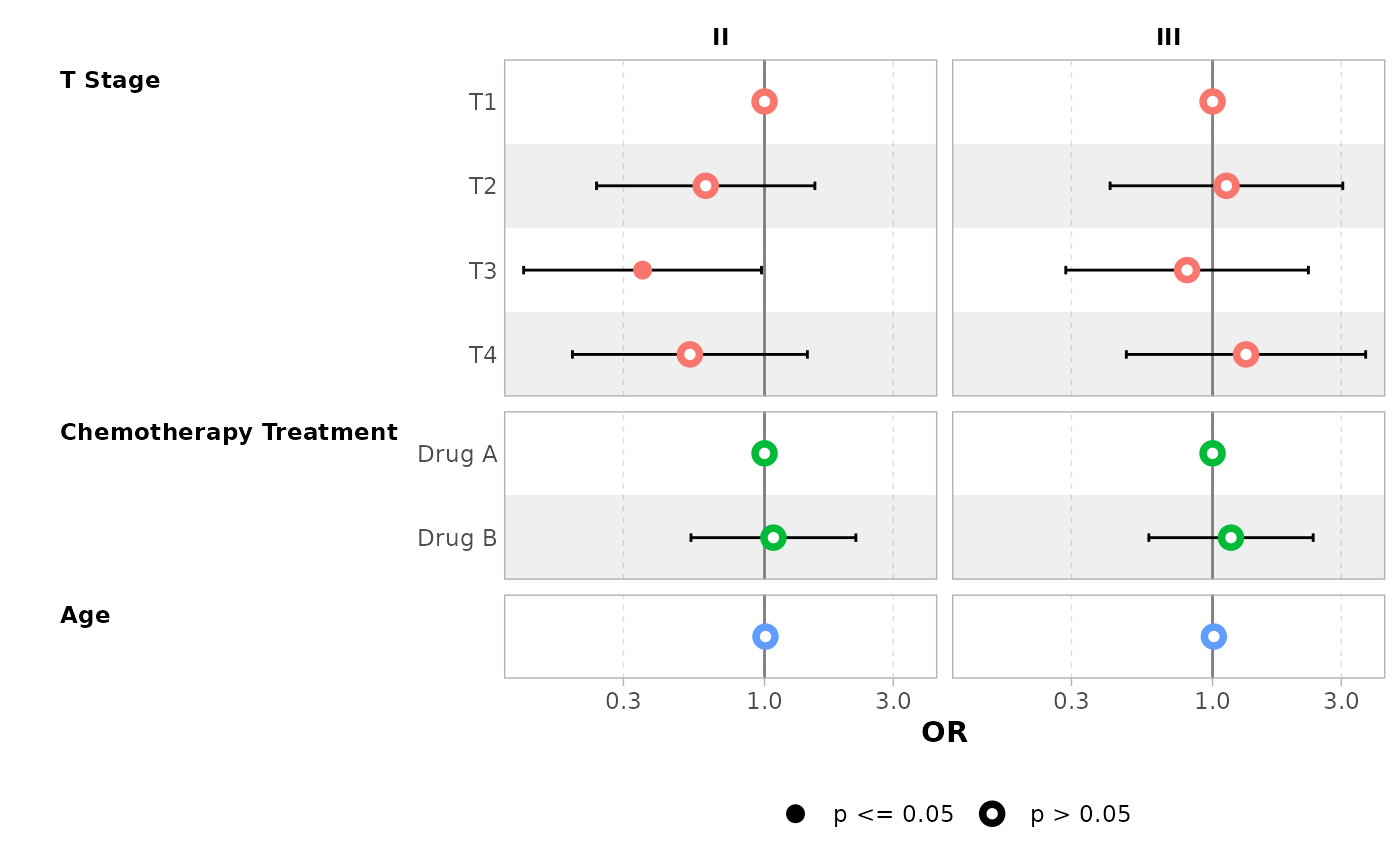ggcoef_multinom(mod, type = "faceted")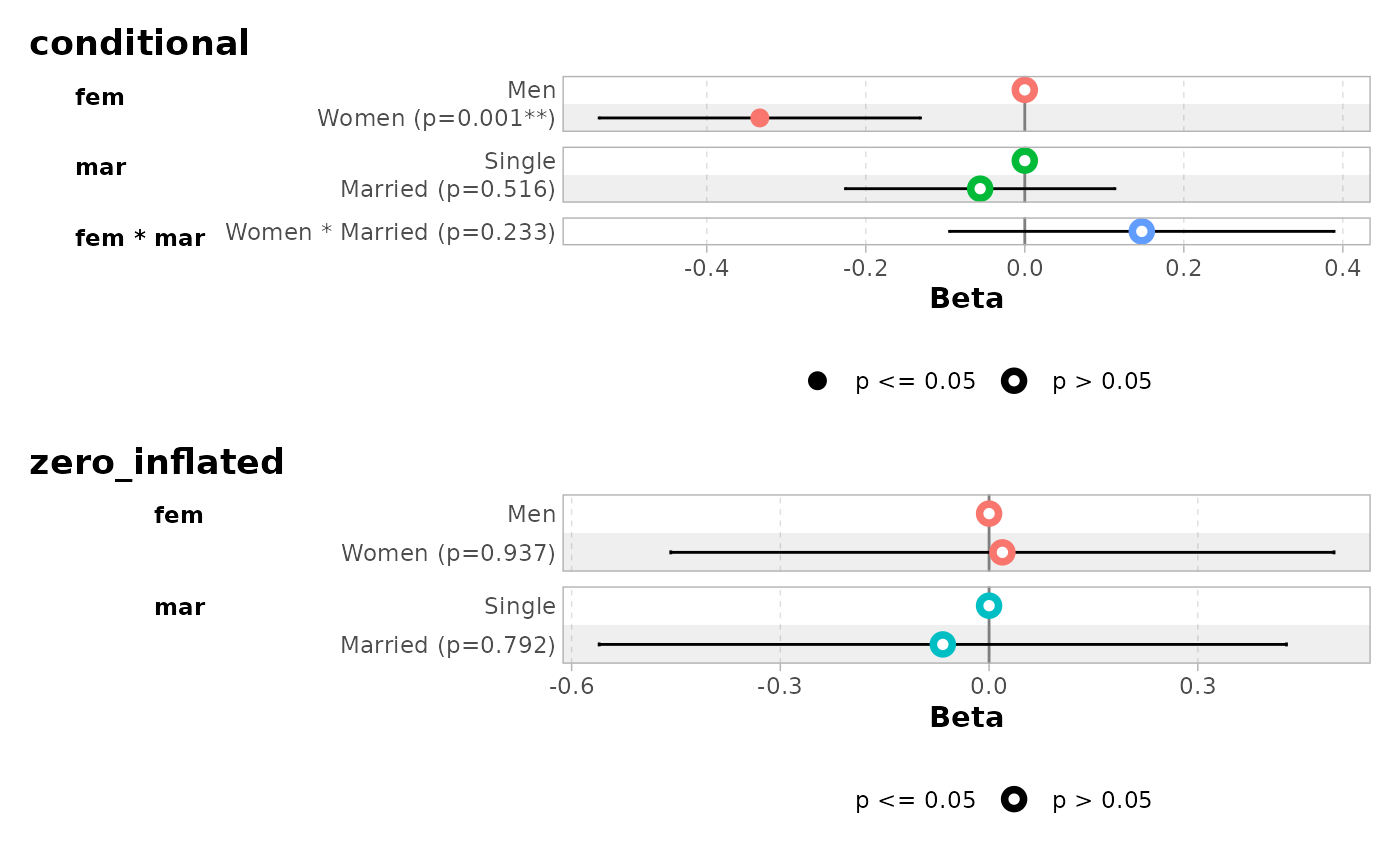ggcoef_multinom(
mod,
type = "faceted",
y.level_label = c("versicolor" = "versicolor\n(ref: setosa)")
)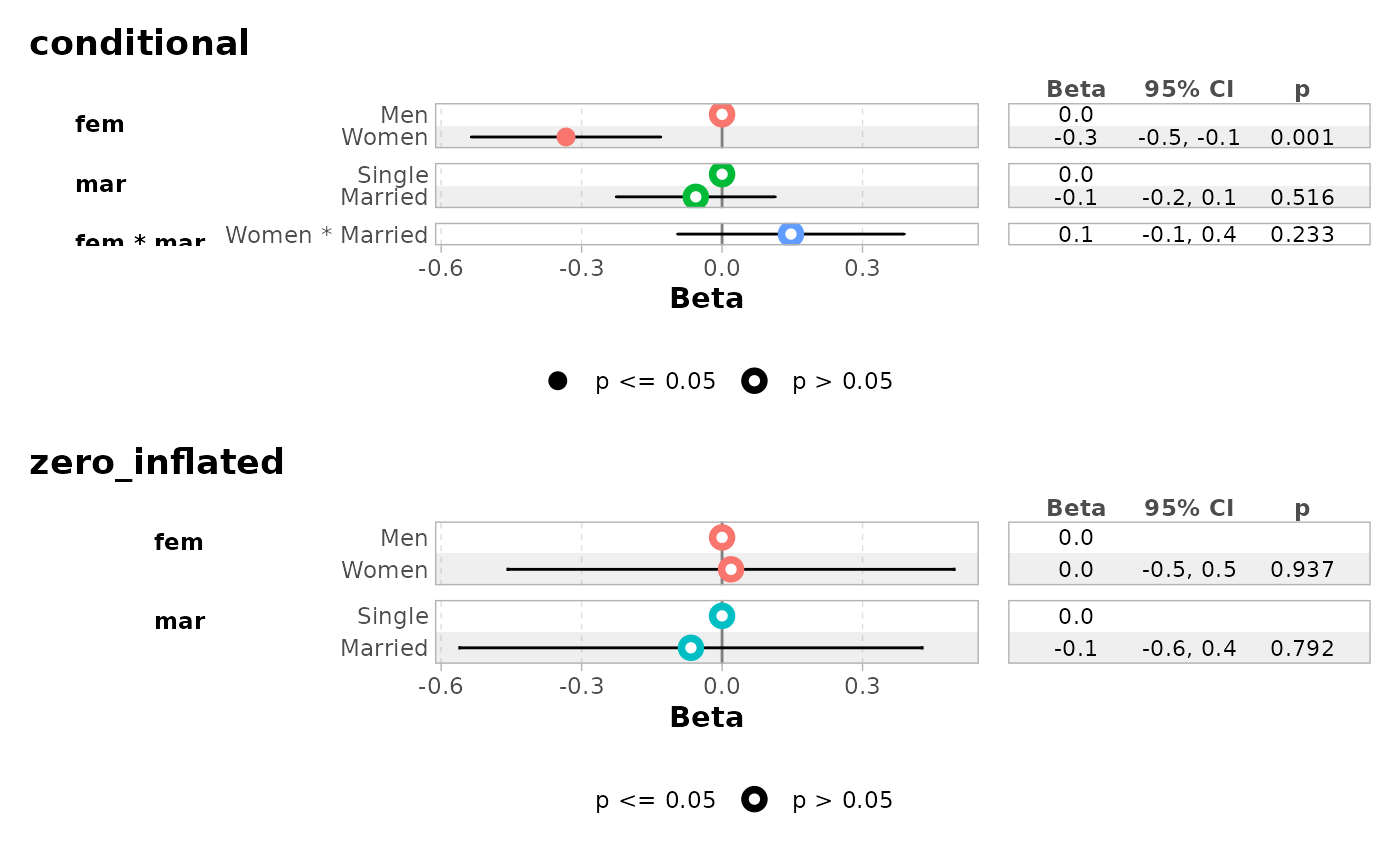# }
# \donttest{
library(pscl)
#> Classes and Methods for R developed in the
#> Political Science Computational Laboratory
#> Department of Political Science
#> Stanford University
#> Simon Jackman
#> hurdle and zeroinfl functions by Achim Zeileis
data("bioChemists", package = "pscl")
mod <- zeroinfl(art ~ fem * mar | fem + mar, data = bioChemists)
ggcoef_multicomponents(mod)
#> ℹ <zeroinfl> model detected.
#> ✔ tidy_zeroinfl() used instead.
#> ℹ Add tidy_fun = broom.helpers::tidy_zeroinfl to quiet these messages.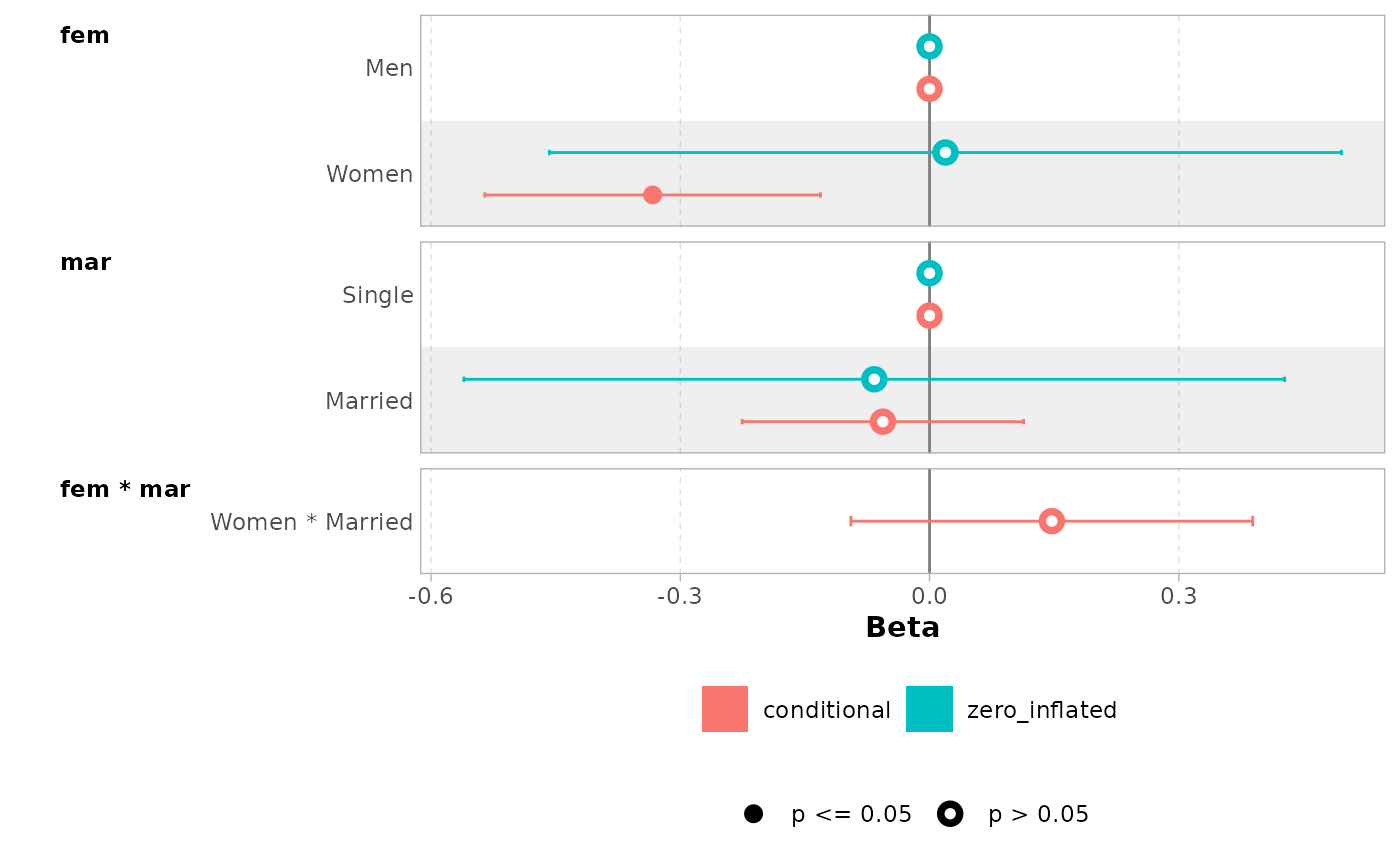ggcoef_multicomponents(mod, type = "f")
#> ℹ <zeroinfl> model detected.
#> ✔ tidy_zeroinfl() used instead.
#> ℹ Add tidy_fun = broom.helpers::tidy_zeroinfl to quiet these messages.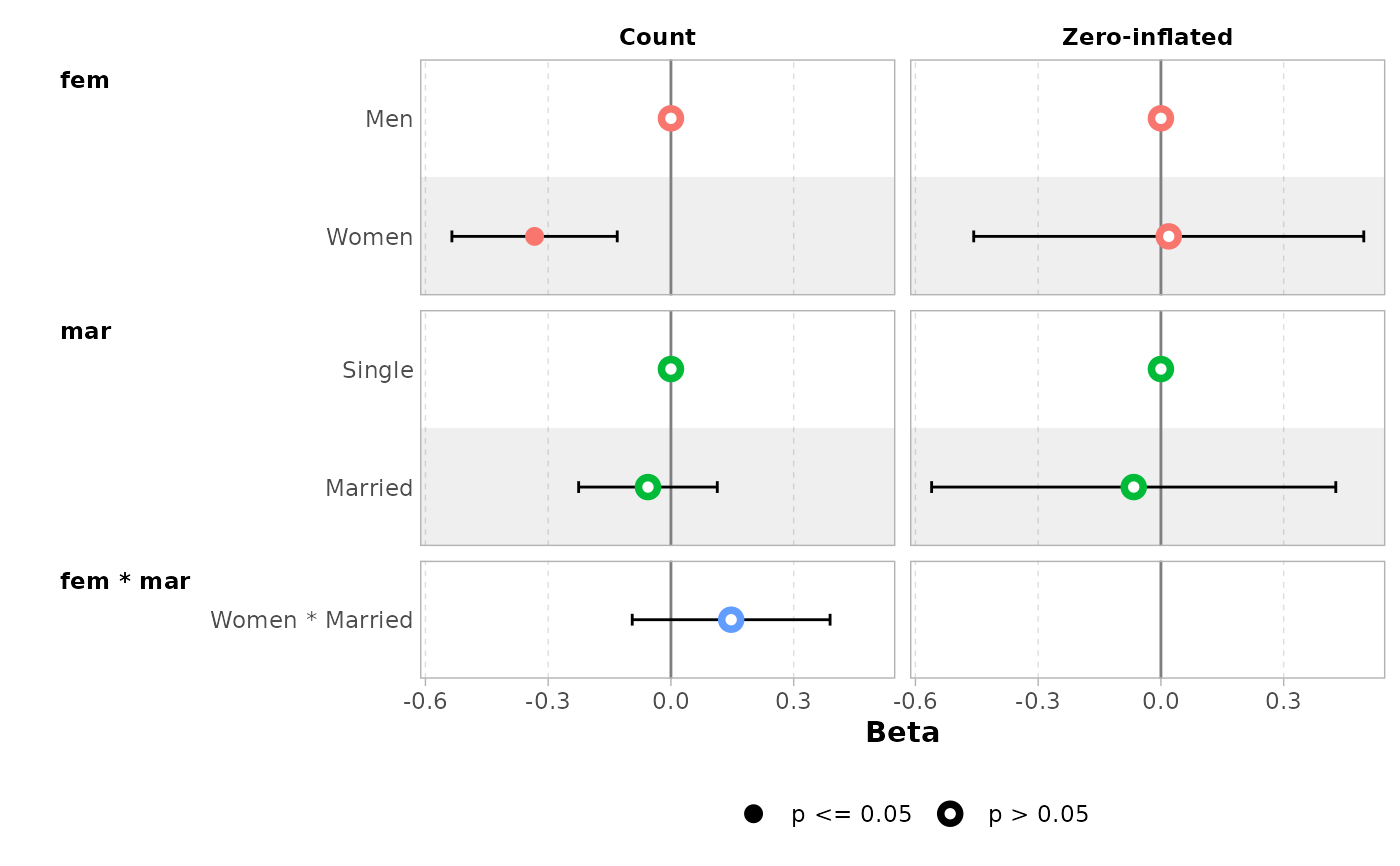ggcoef_multicomponents(mod, type = "t")
#> ℹ <zeroinfl> model detected.
#> ✔ tidy_zeroinfl() used instead.
#> ℹ Add tidy_fun = broom.helpers::tidy_zeroinfl to quiet these messages.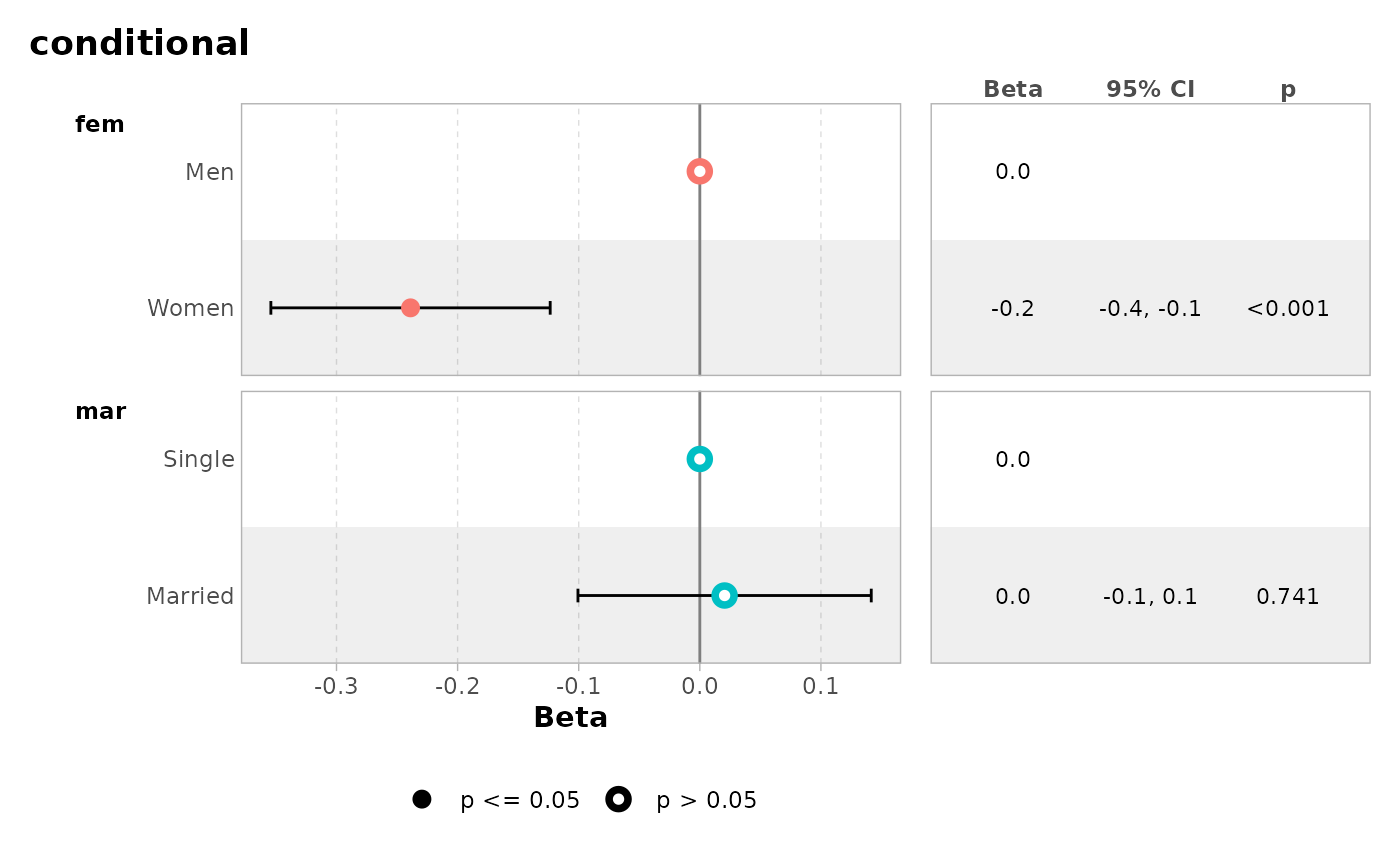ggcoef_multicomponents(
mod,
type = "t",
component_label = c(conditional = "Count", zero_inflated = "Zero-inflated")
)
#> ℹ <zeroinfl> model detected.
#> ✔ tidy_zeroinfl() used instead.
#> ℹ Add tidy_fun = broom.helpers::tidy_zeroinfl to quiet these messages.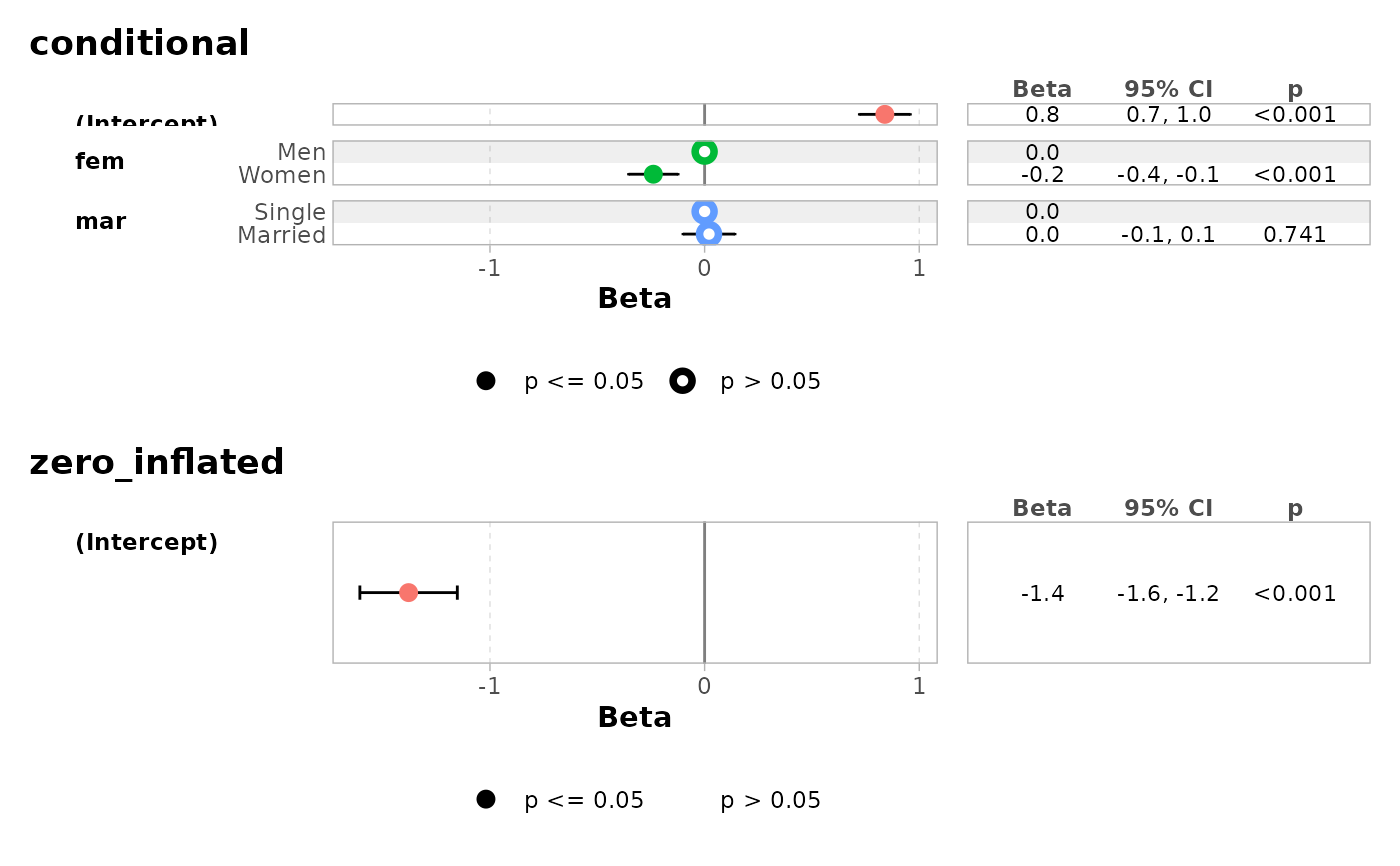mod2 <- zeroinfl(art ~ fem + mar | 1, data = bioChemists)
ggcoef_multicomponents(mod2, type = "t")
#> ℹ <zeroinfl> model detected.
#> ✔ tidy_zeroinfl() used instead.
#> ℹ Add tidy_fun = broom.helpers::tidy_zeroinfl to quiet these messages.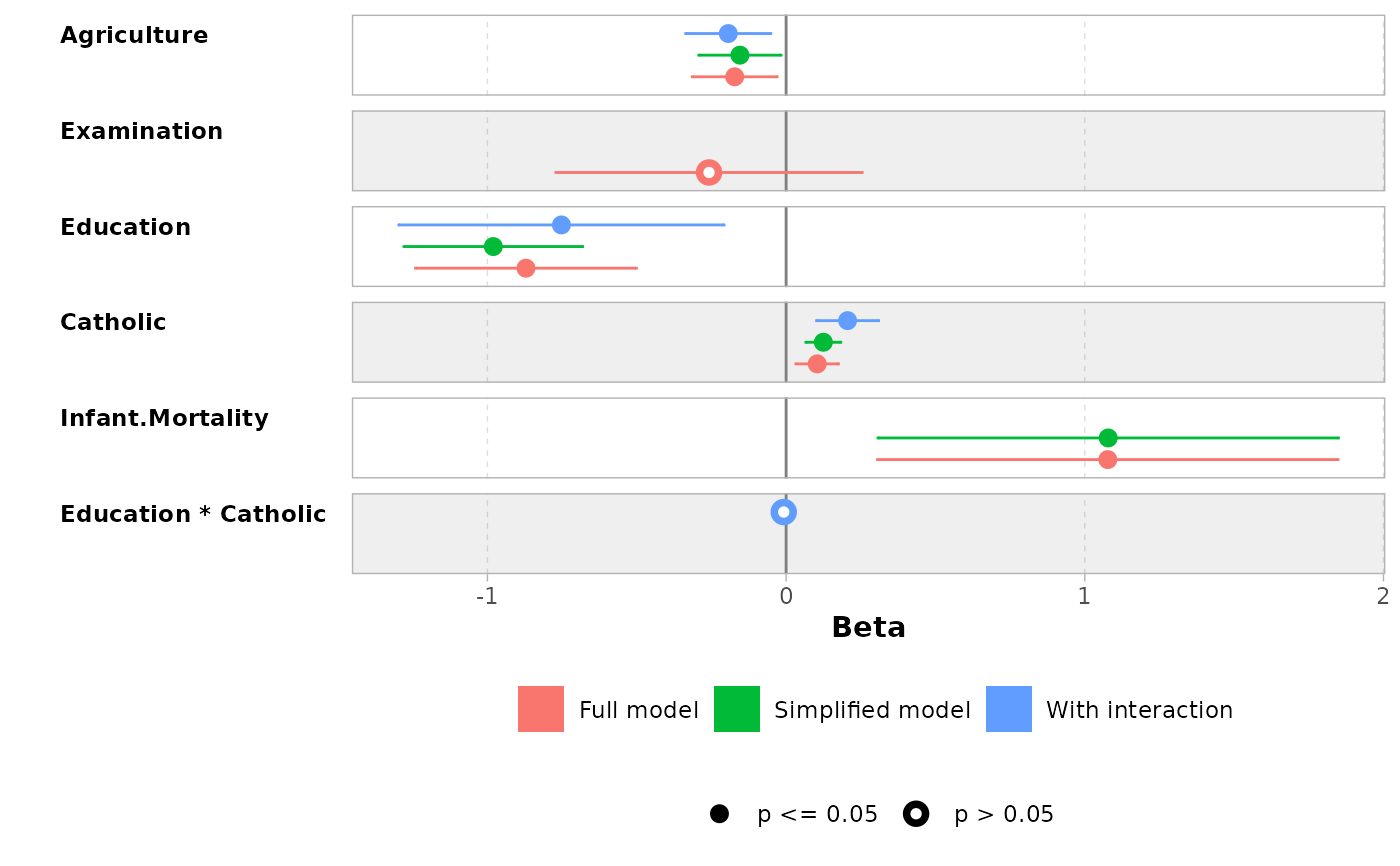# }